# Madhya Pradesh Board Question Paper for Class 10th Maths 2016 In PDF

## MP Board (MPBSE) Question Paper 2016 Class 10th Mathematics For English Medium with Solutions – Free Download

MP Board Question Paper 2016 Class 10th Maths with solutions are provided on this page by BYJU’S in downloadable pdf format and also in the text, so that the students are aware of the question paper pattern in the upcoming final exams. Maths Question Paper 2016 Class 10 is also added here, which helps in the exam preparation. Students are able to access all the Madhya Pradesh board previous year maths question papers here. In order to overcome the struggle of completing the paper on time, it is important for the students to practise different types of questions in the previous year papers and succeed in the final board examination.

### MPBSE Class 10th Maths Question Paper With Solutions 2016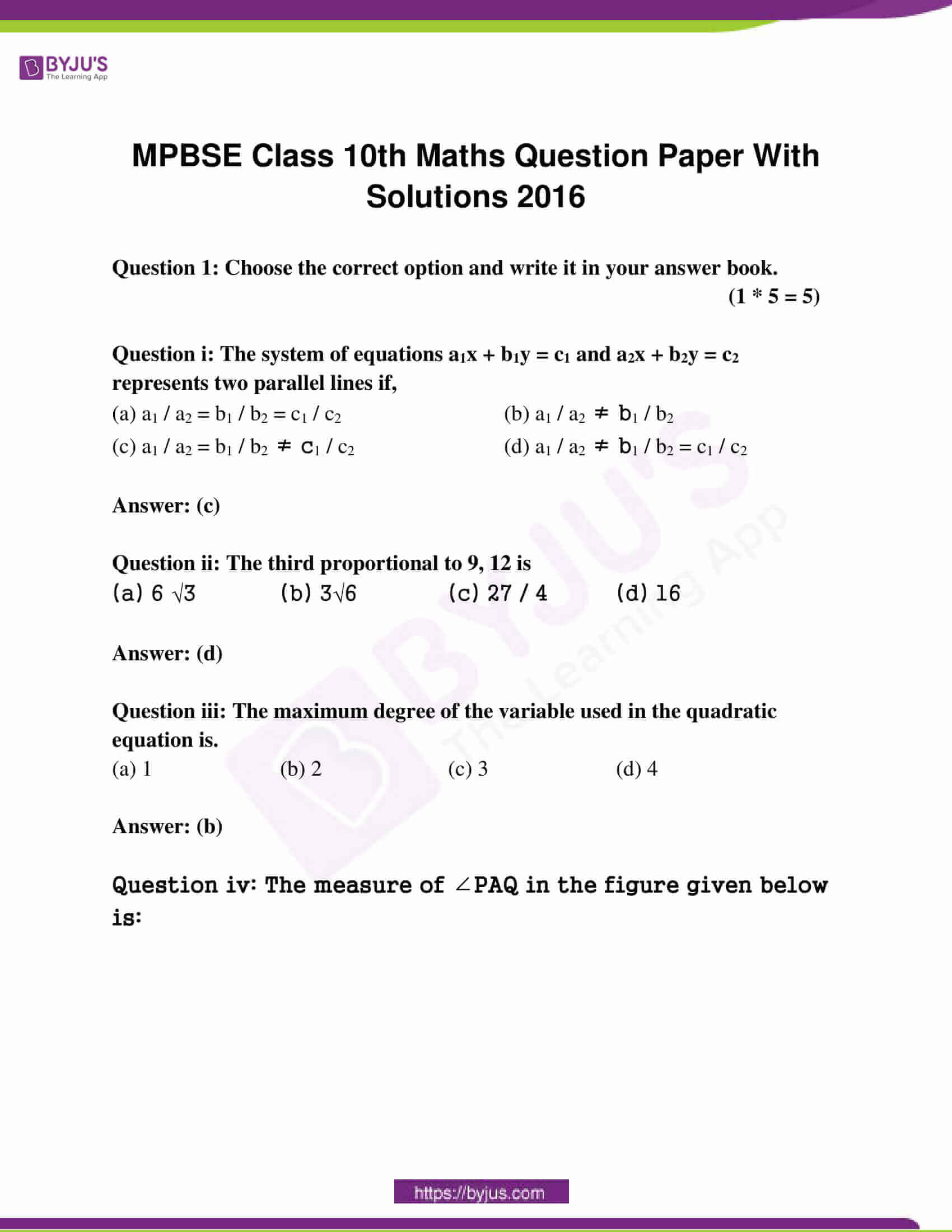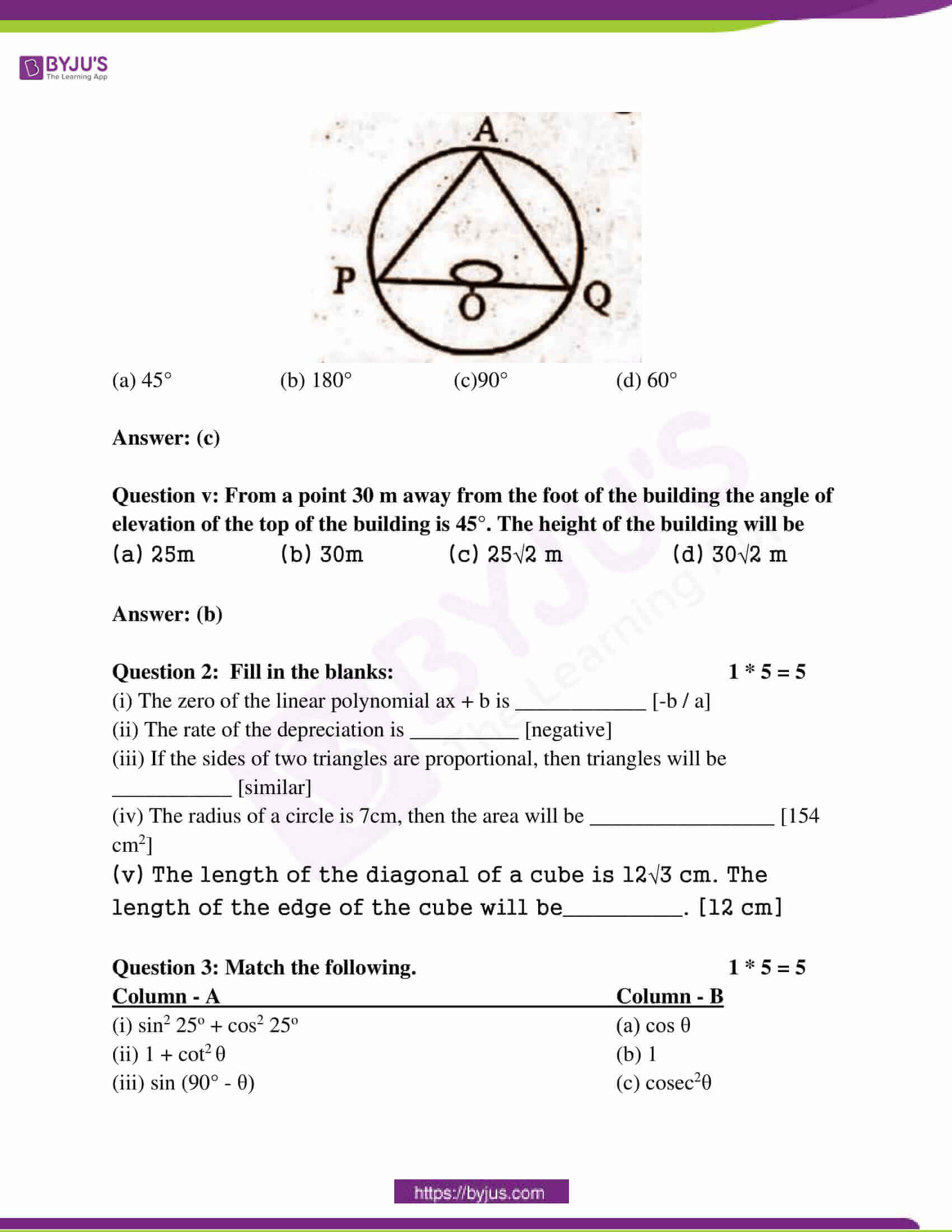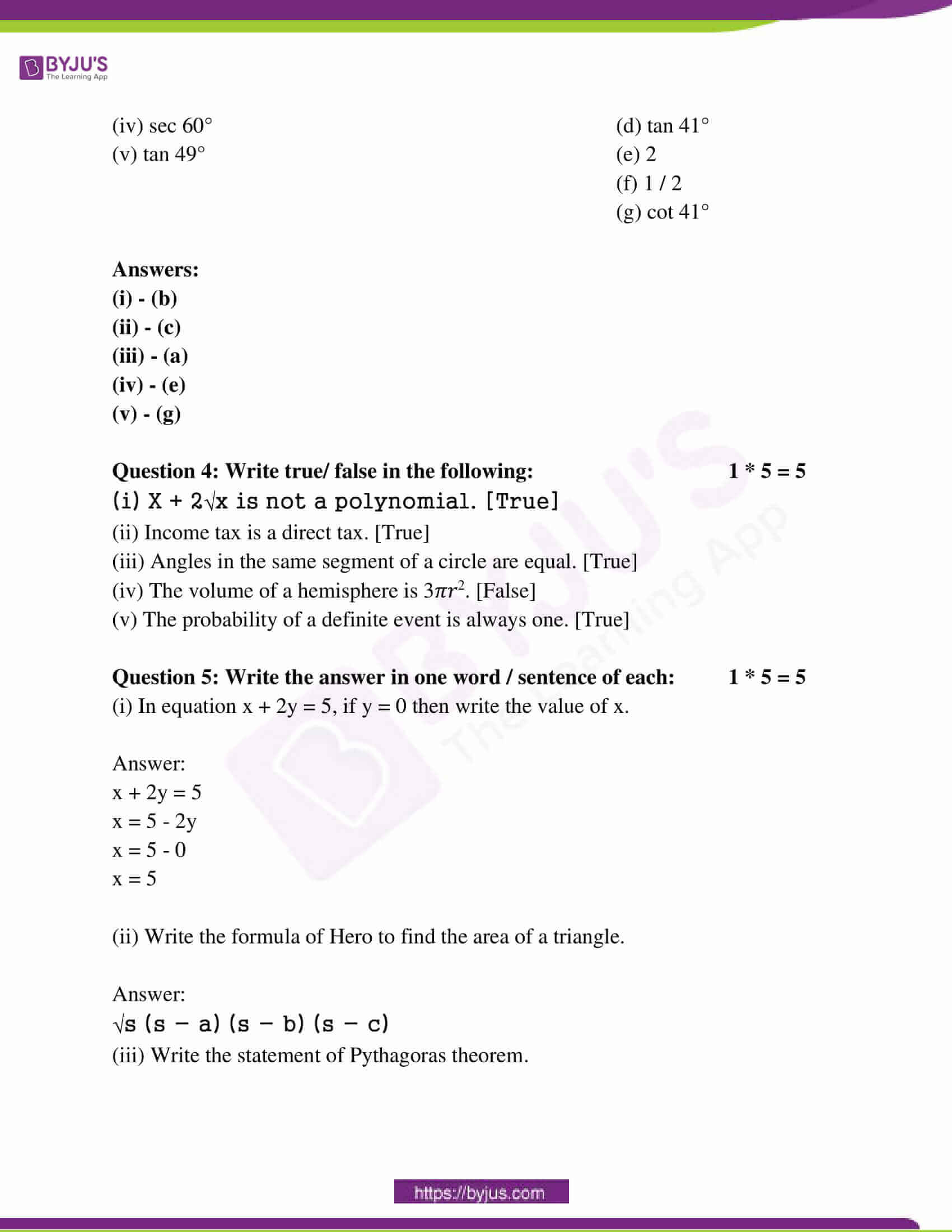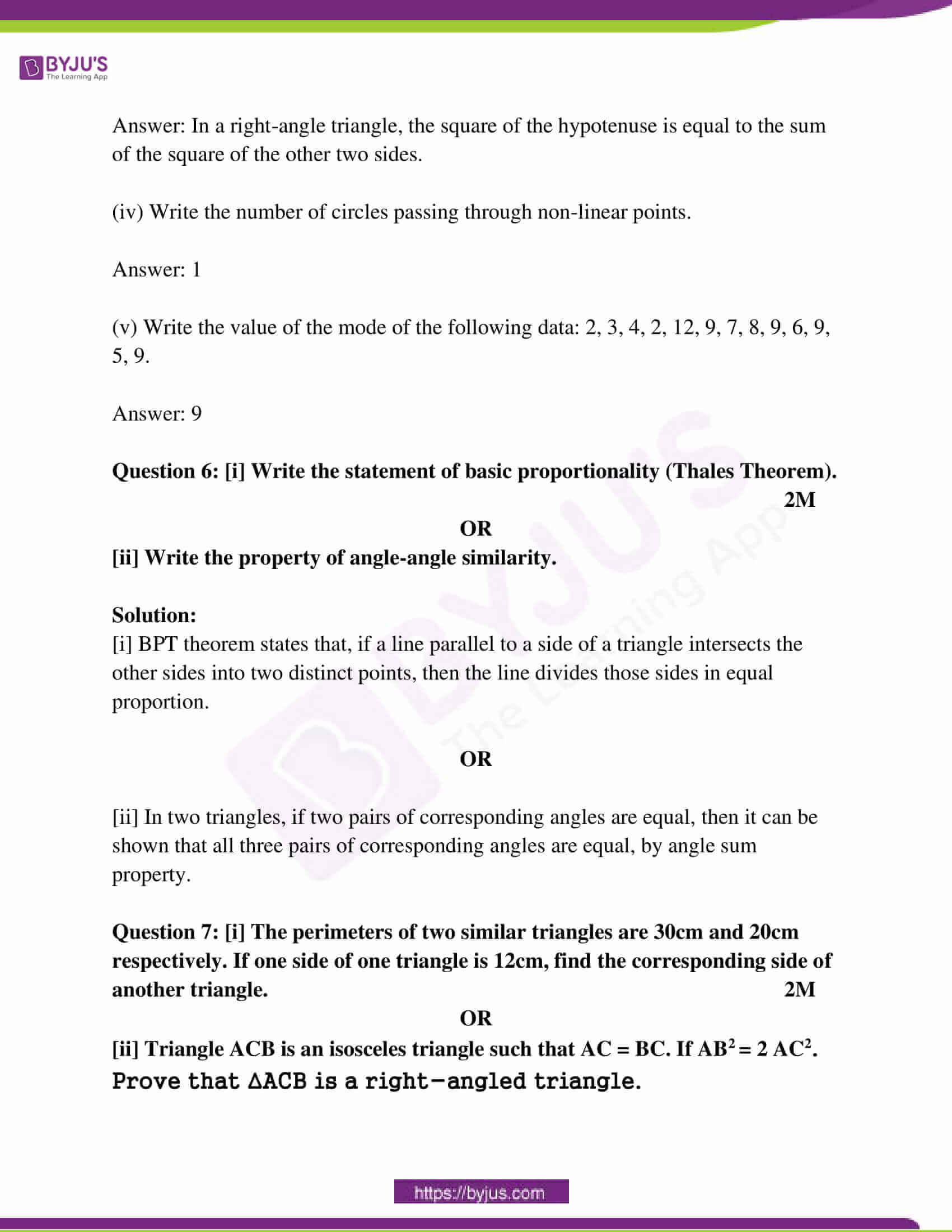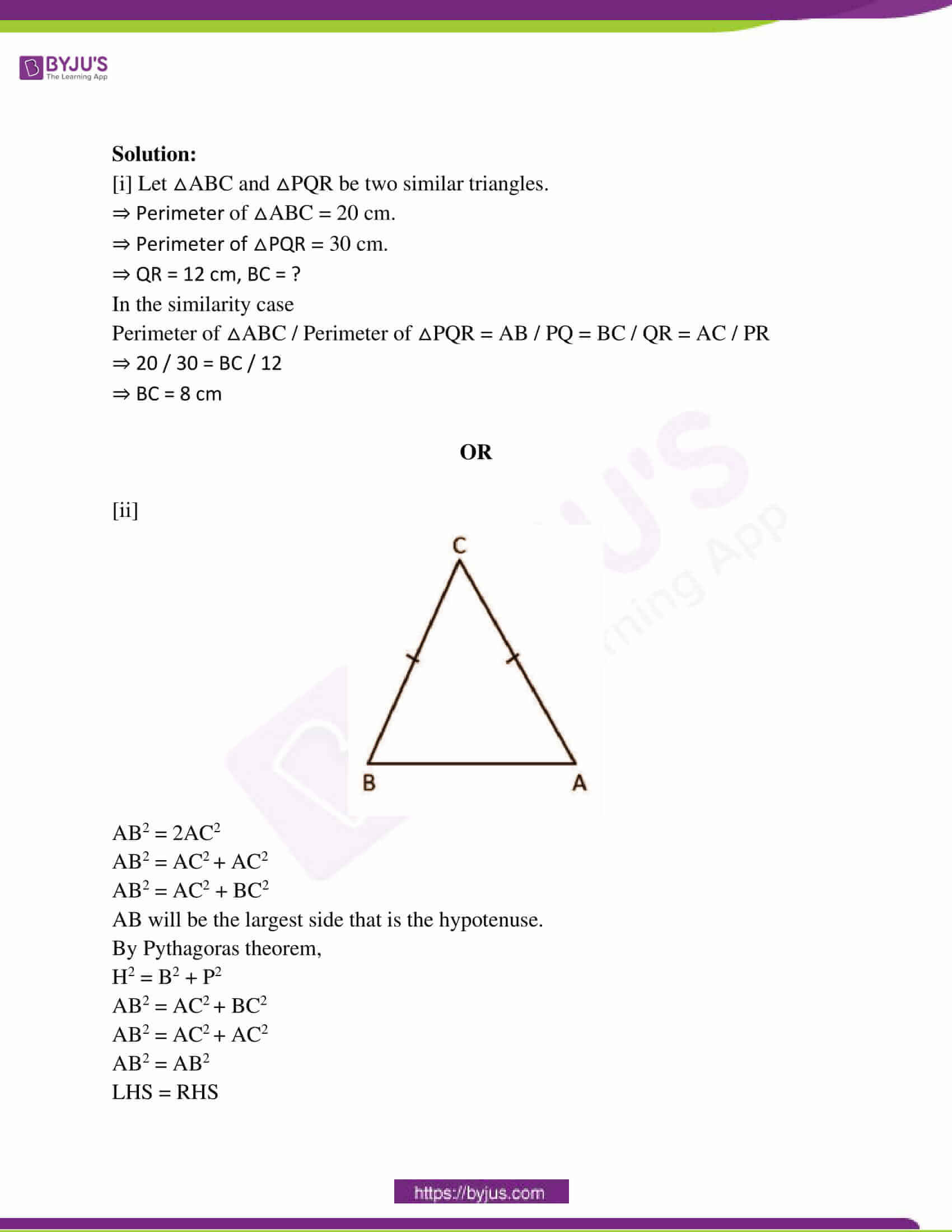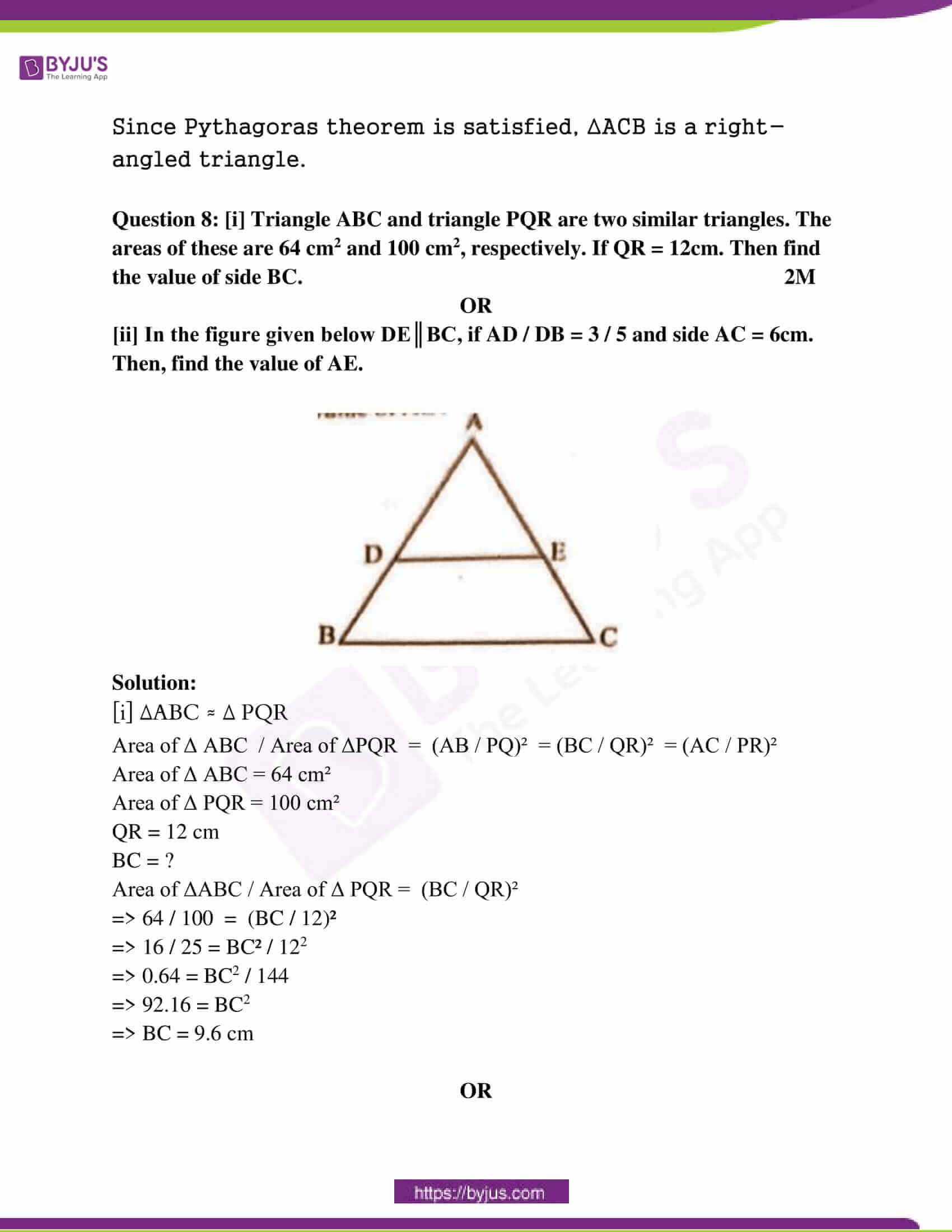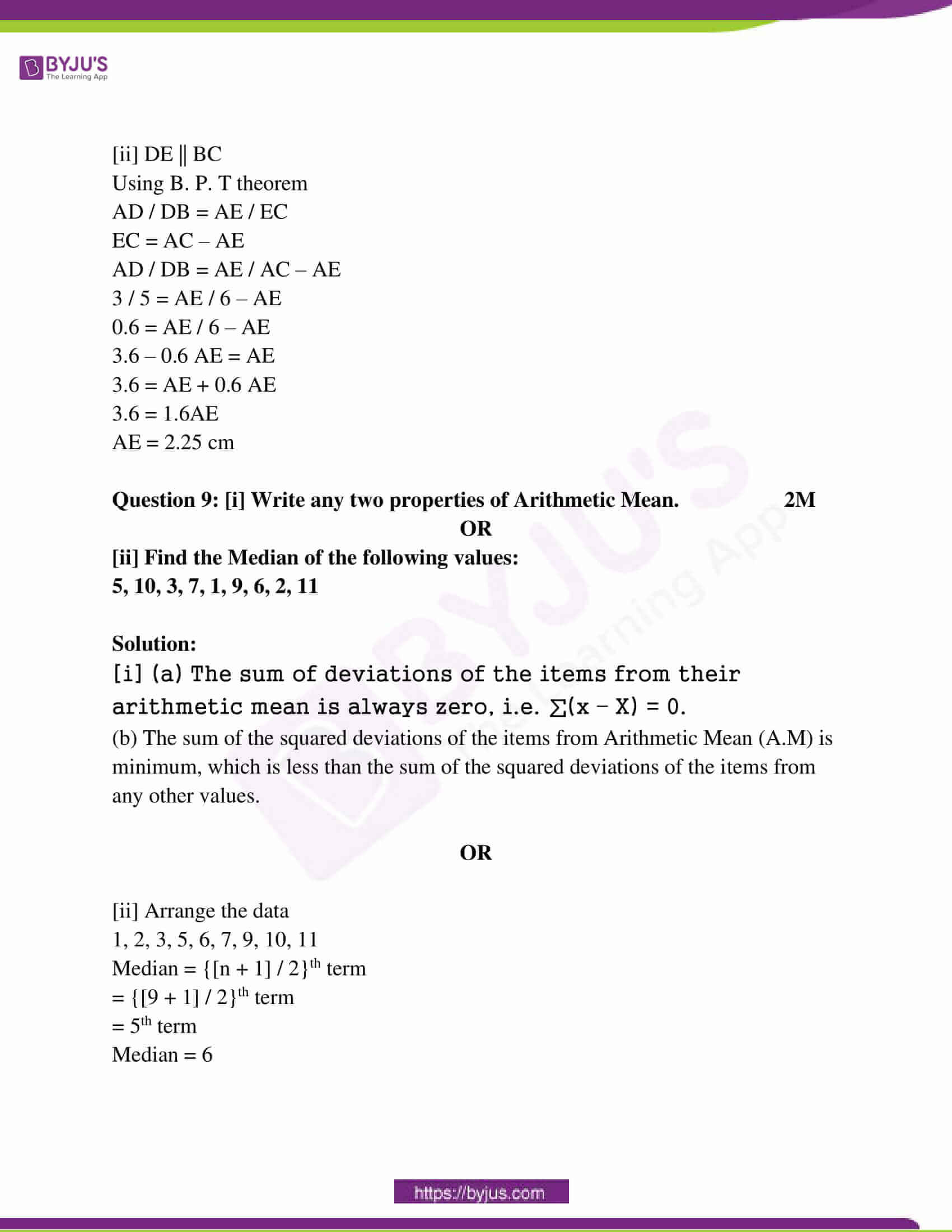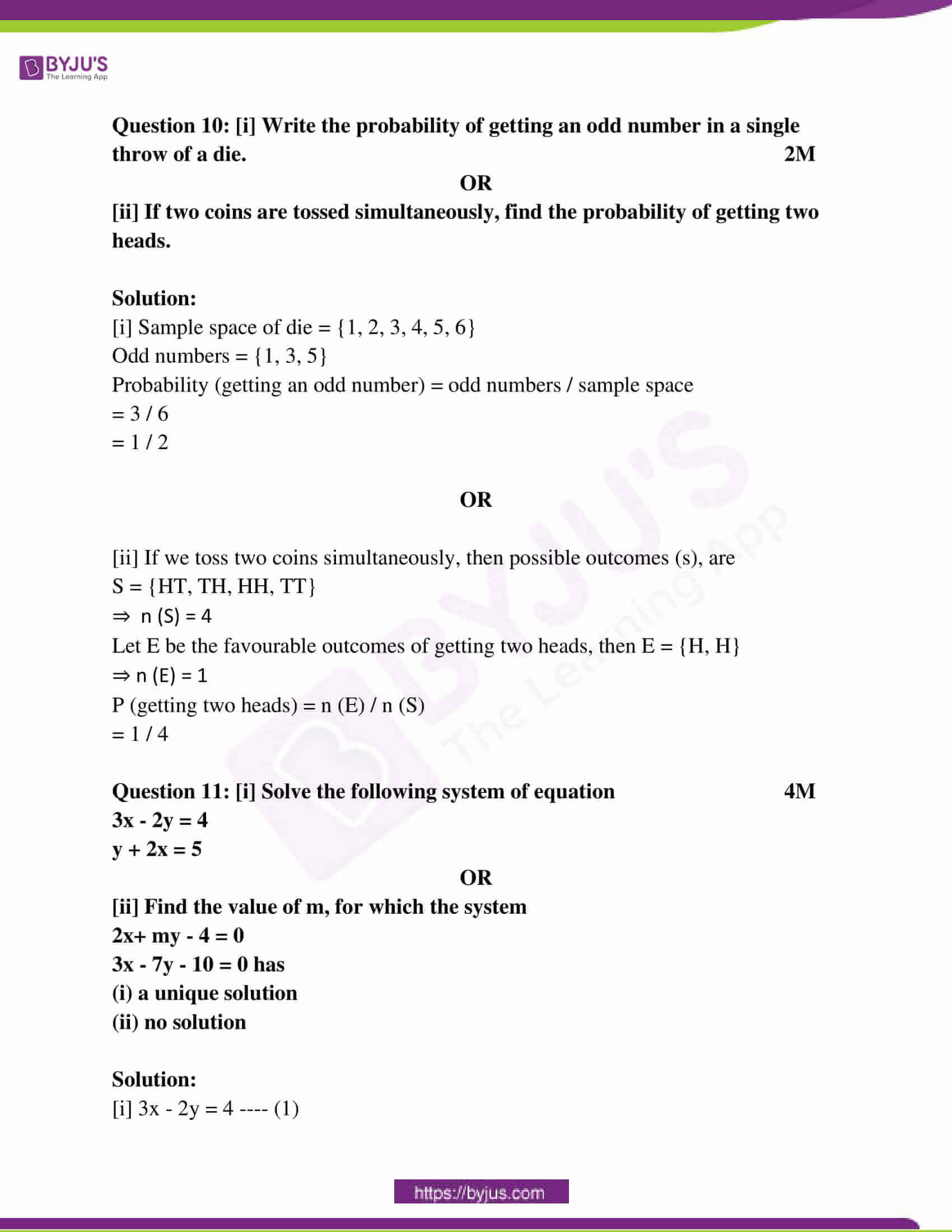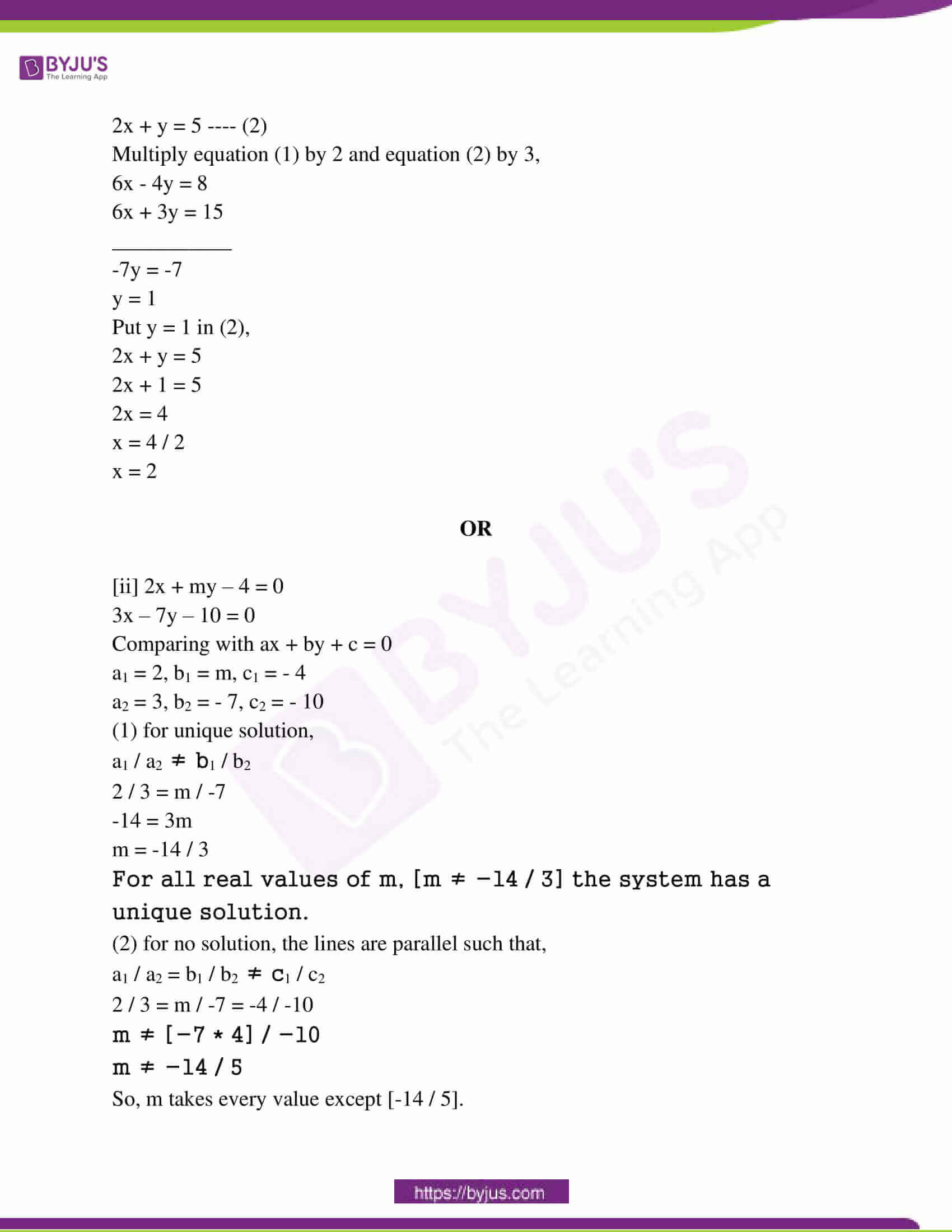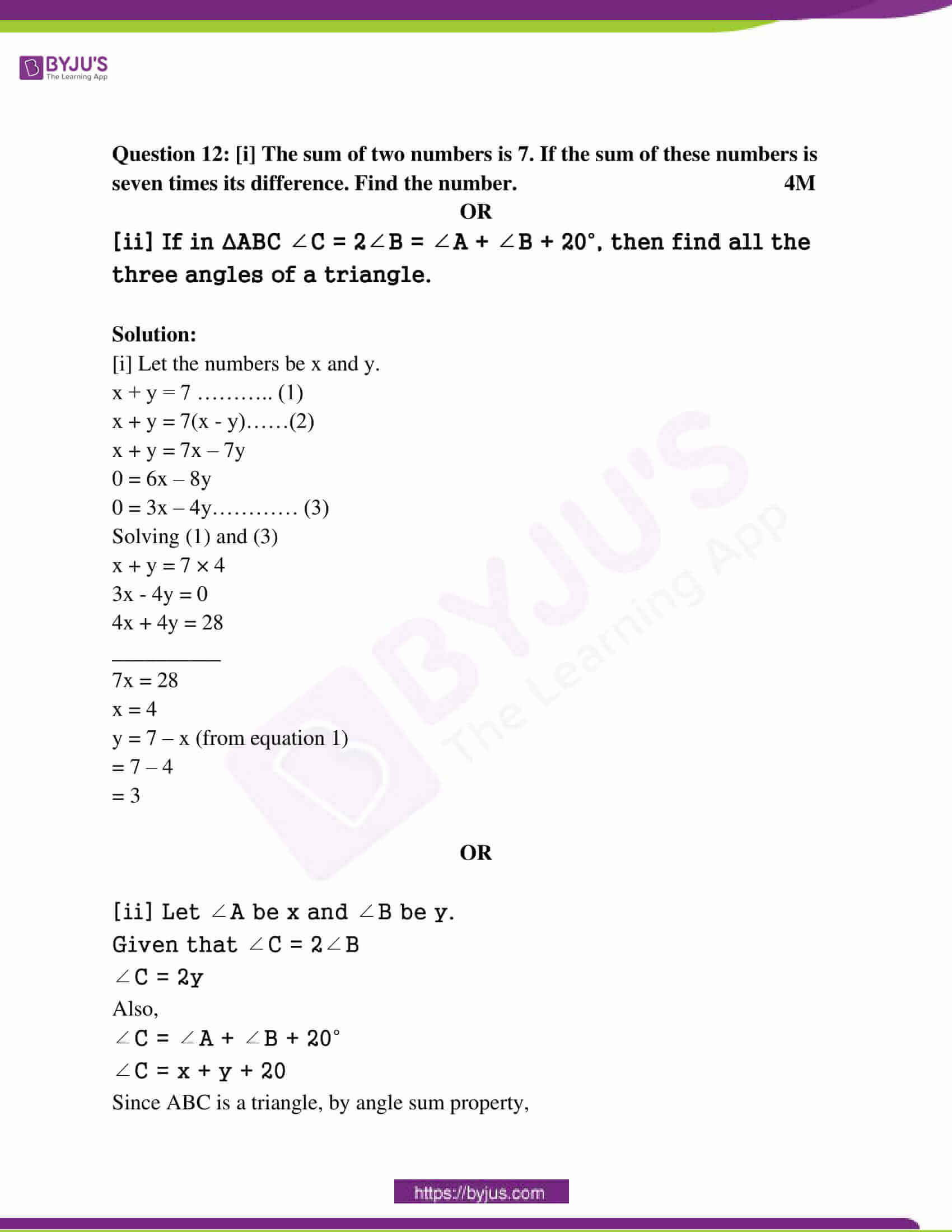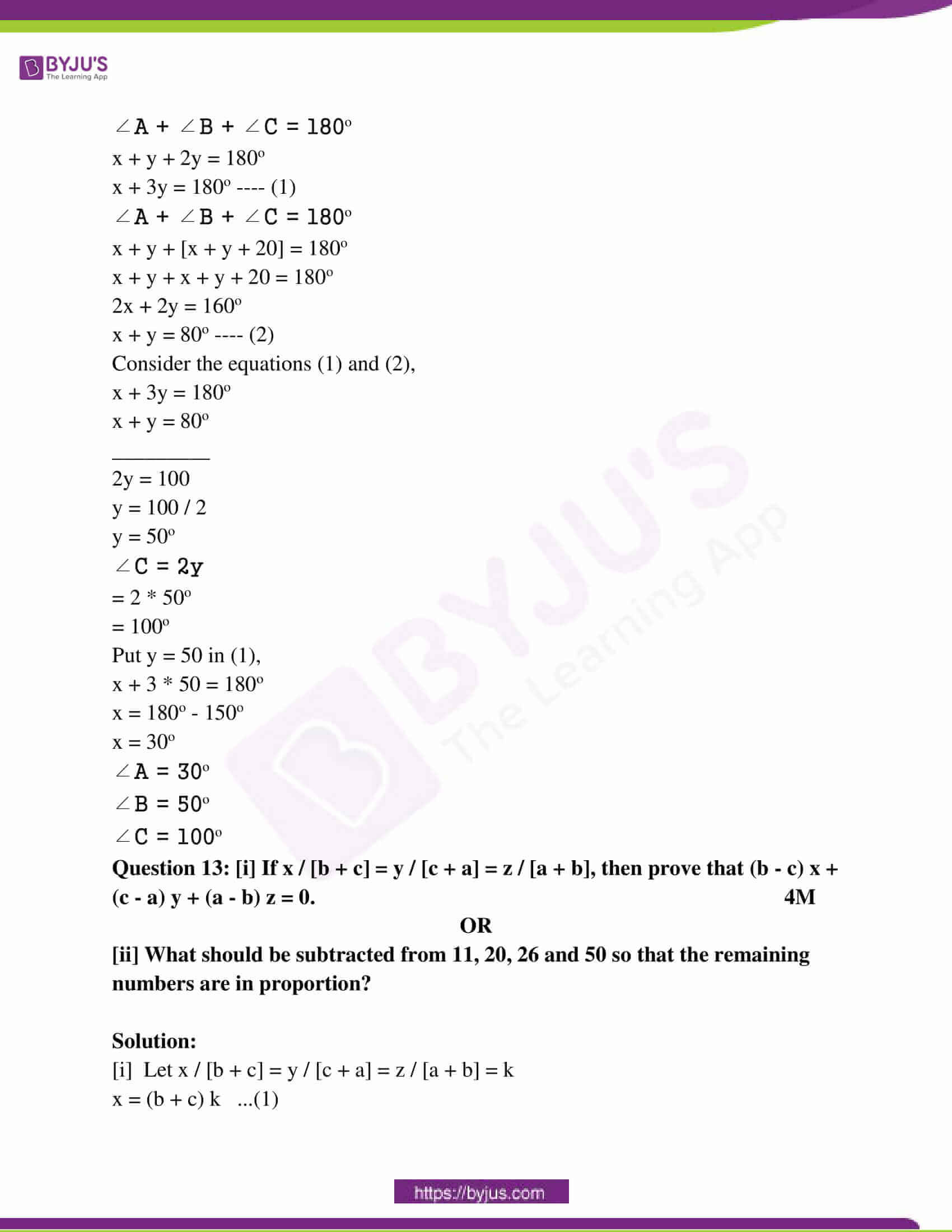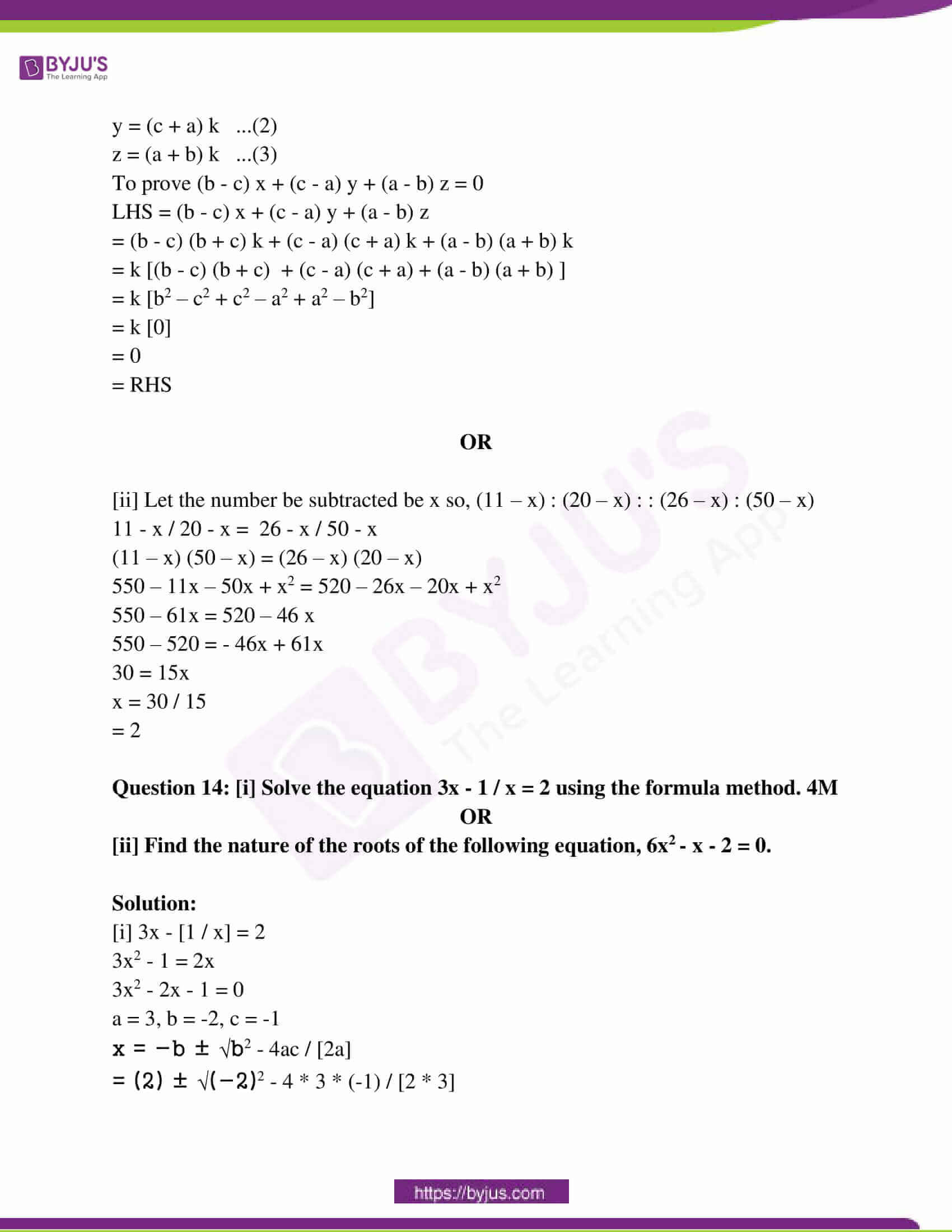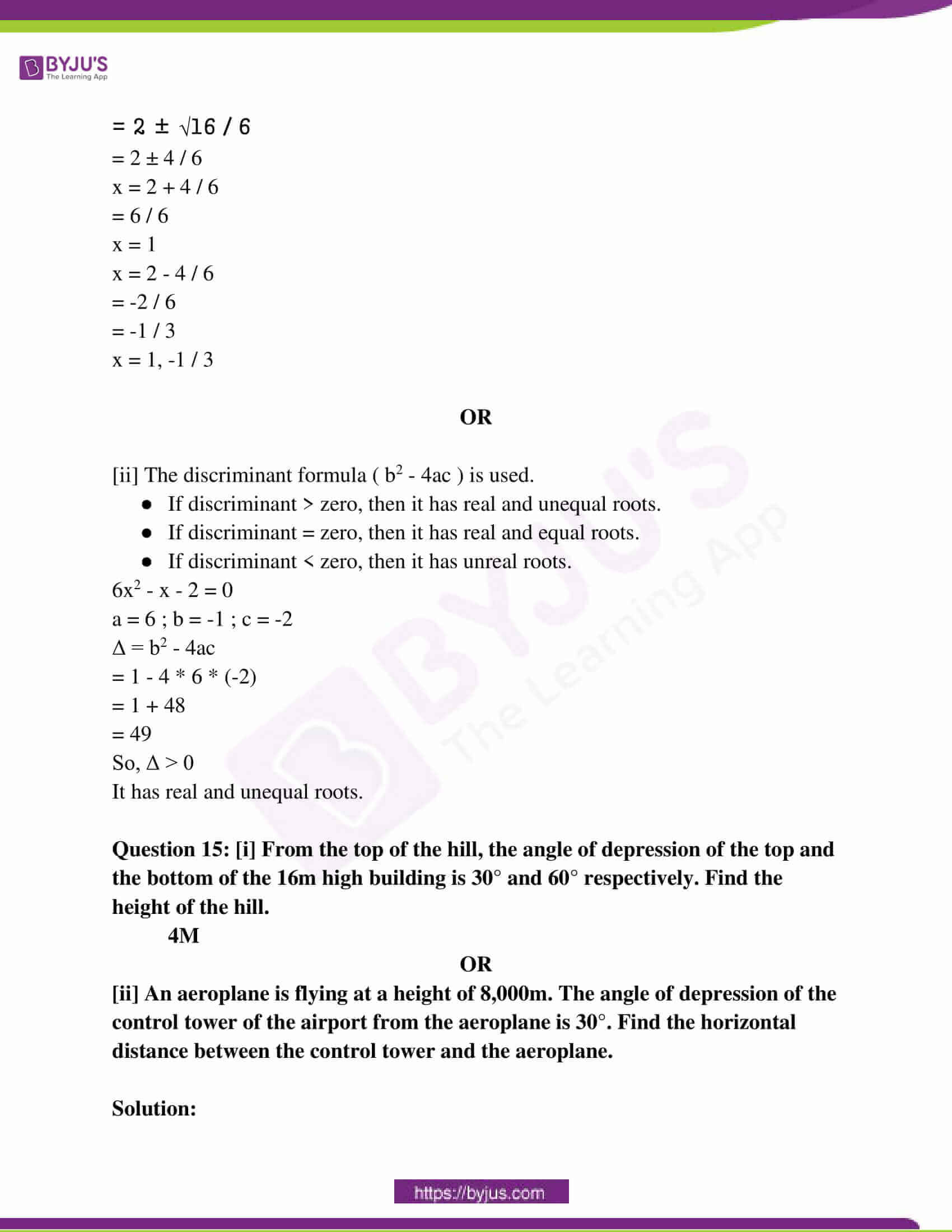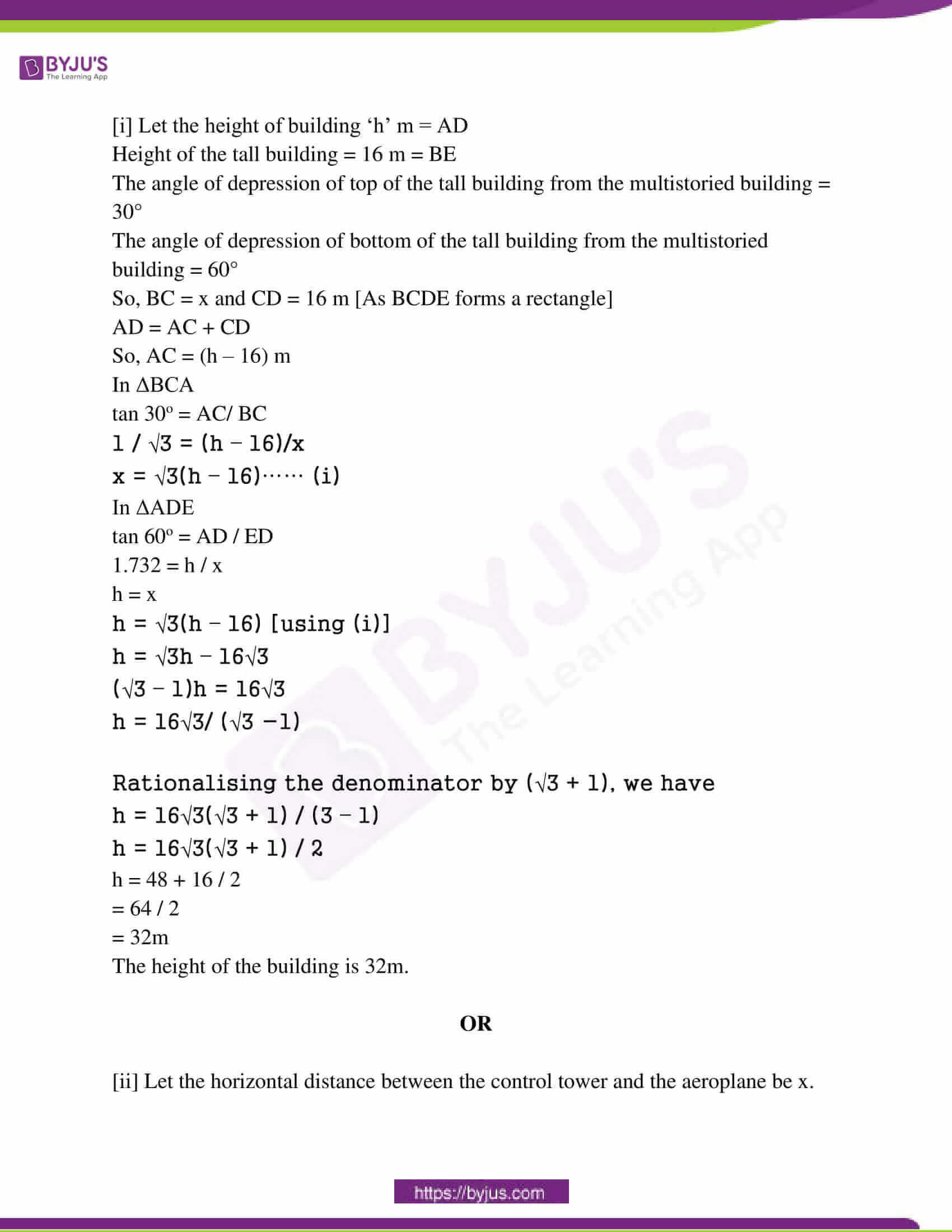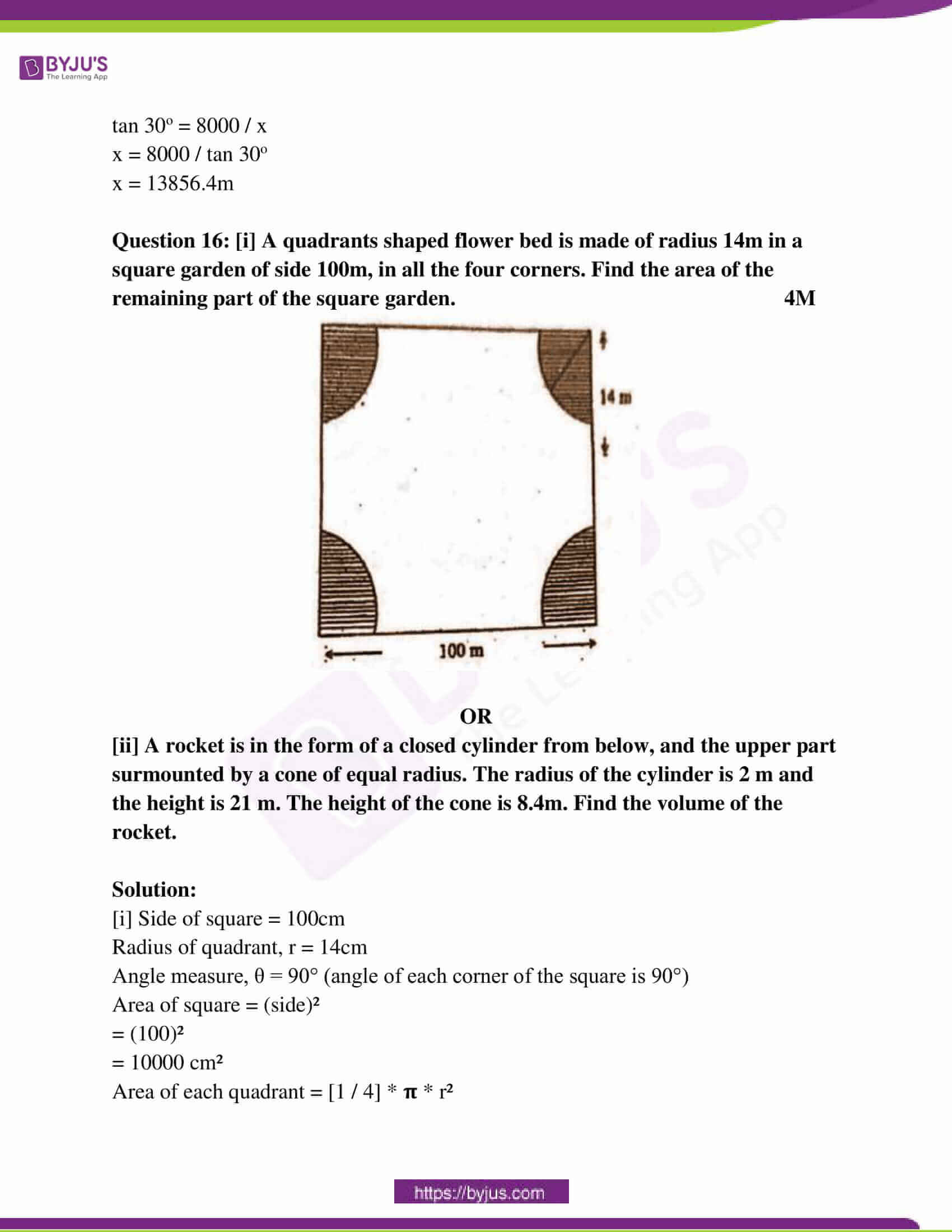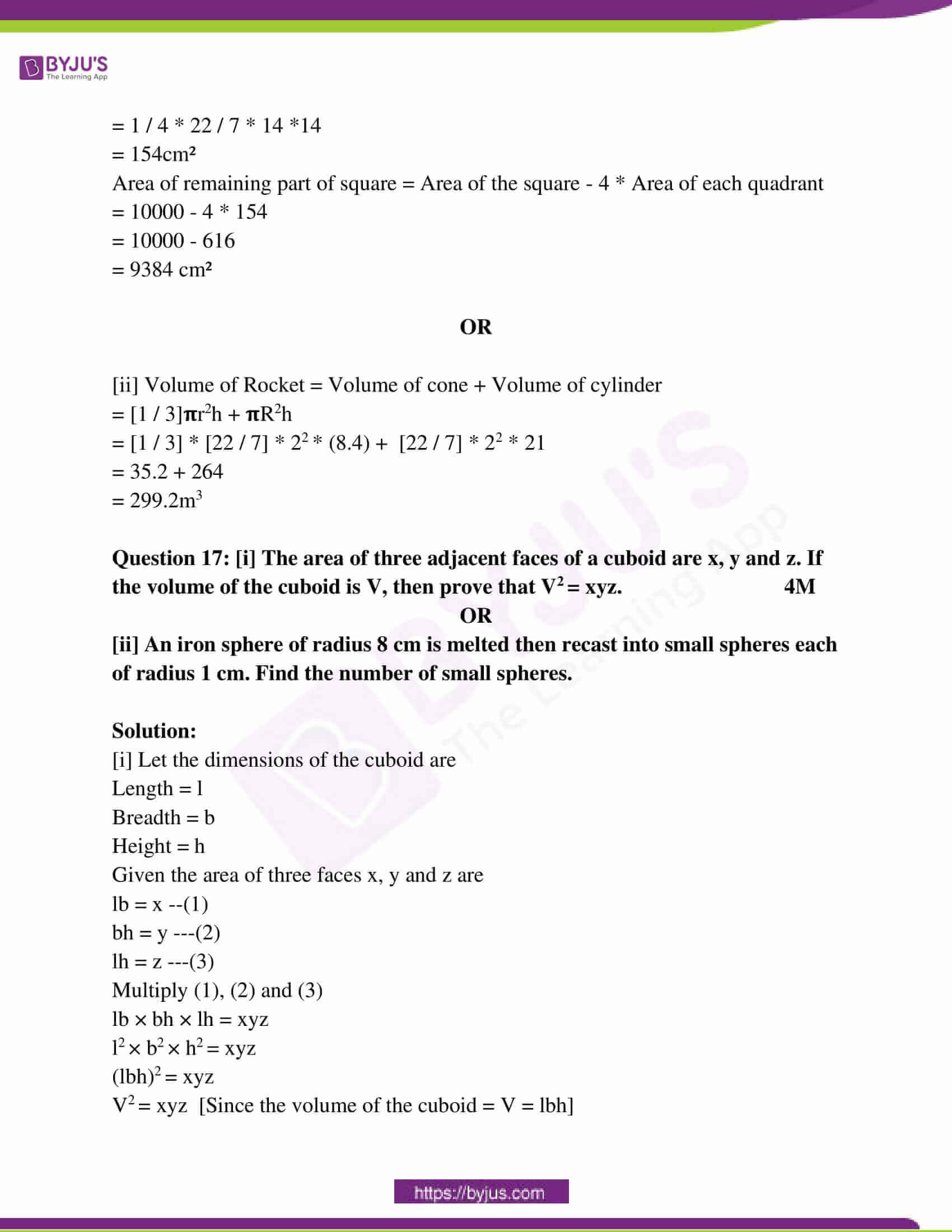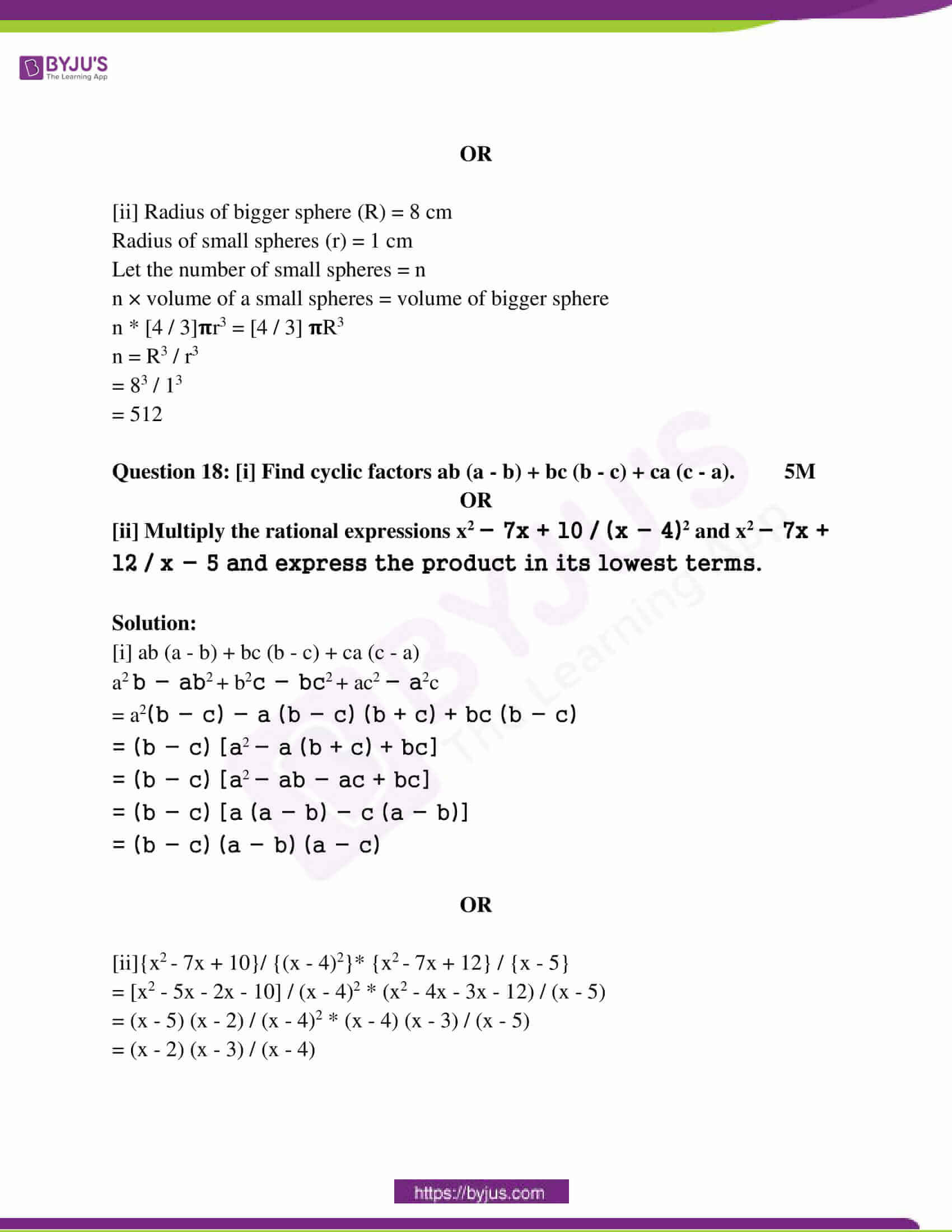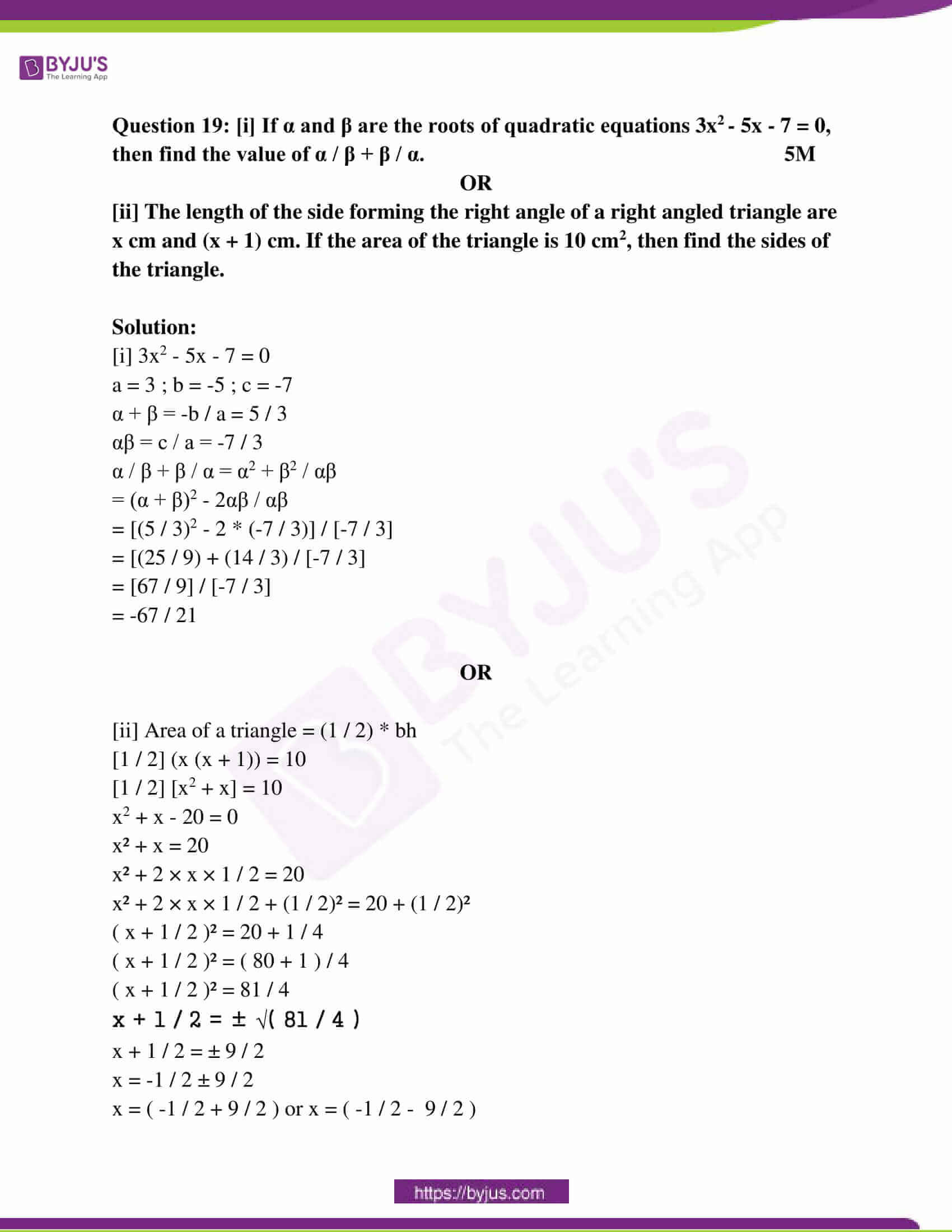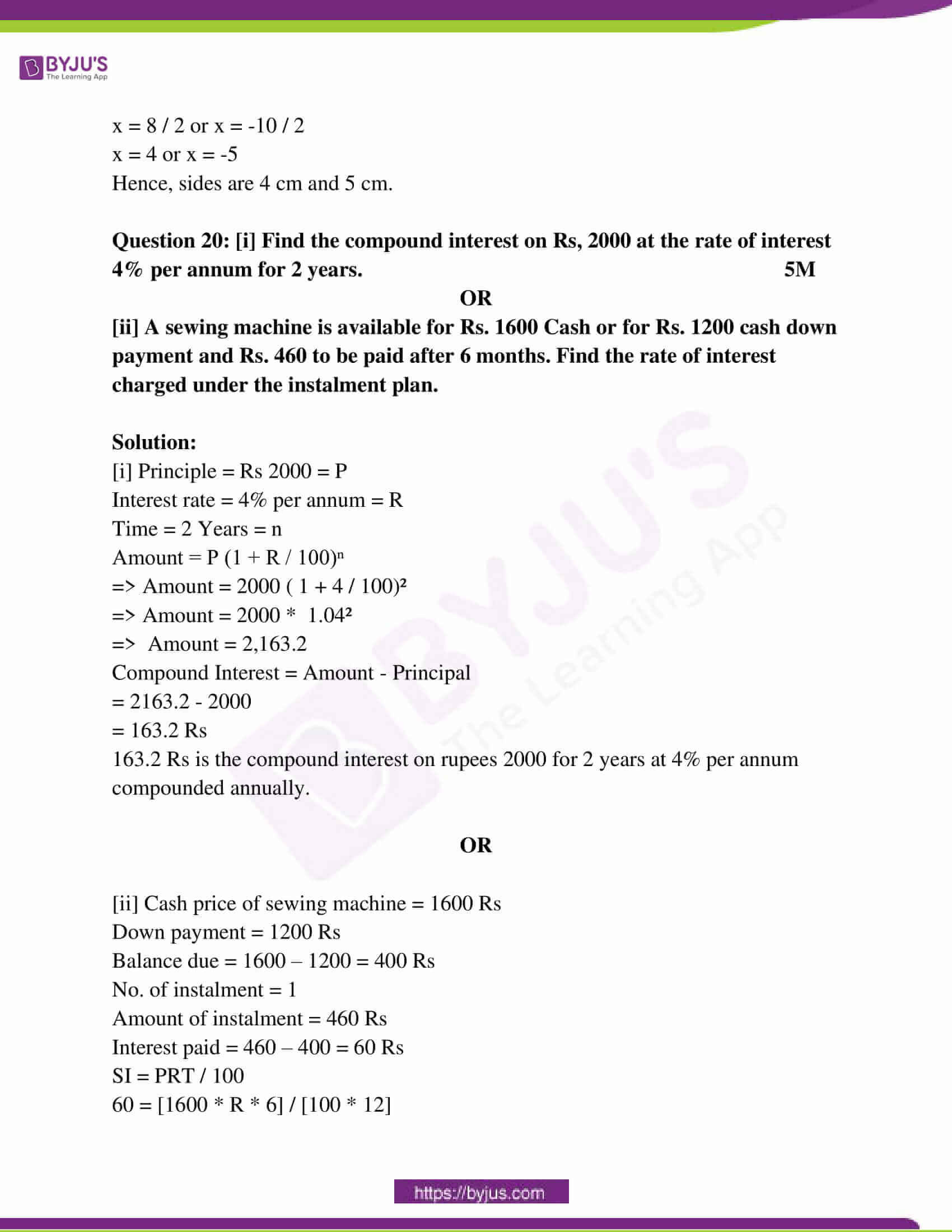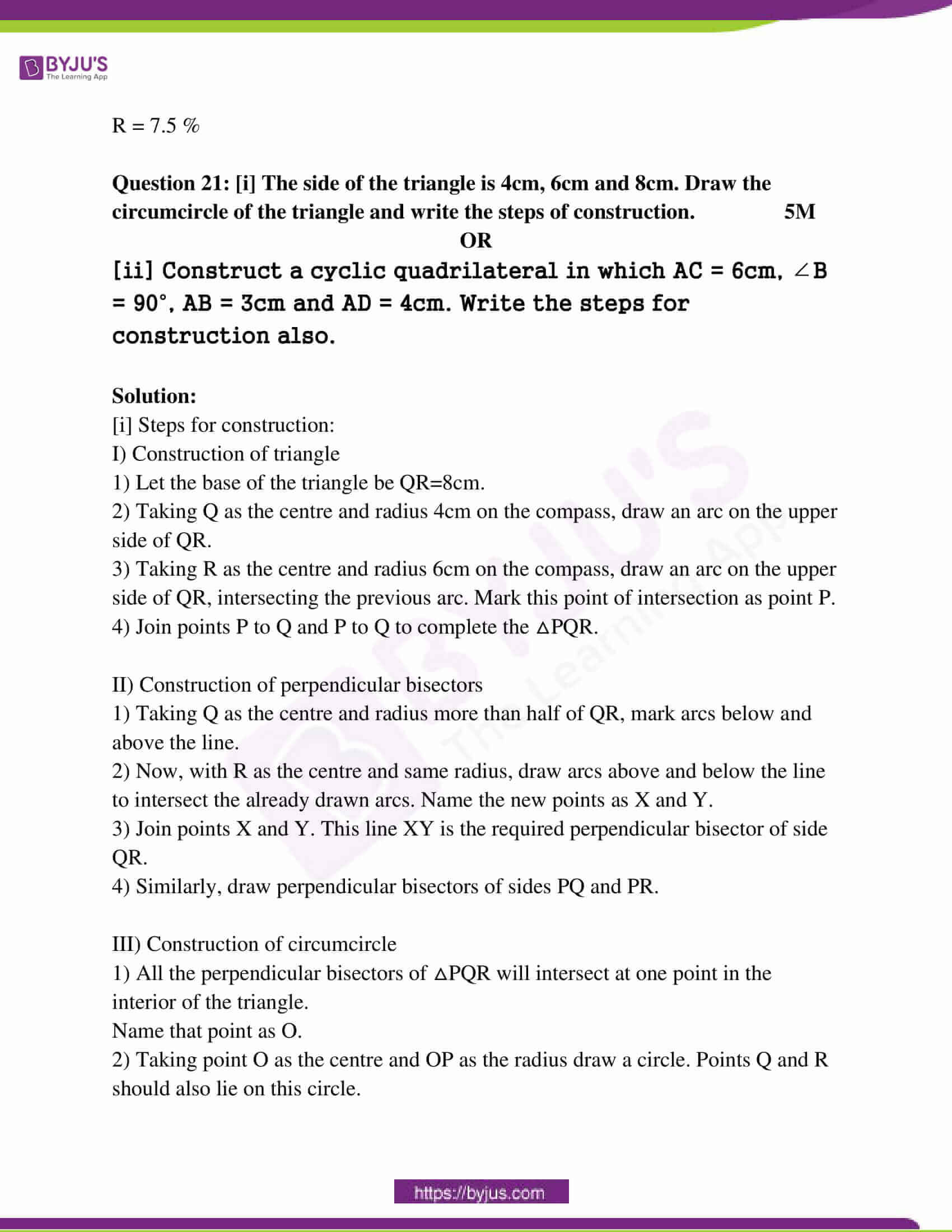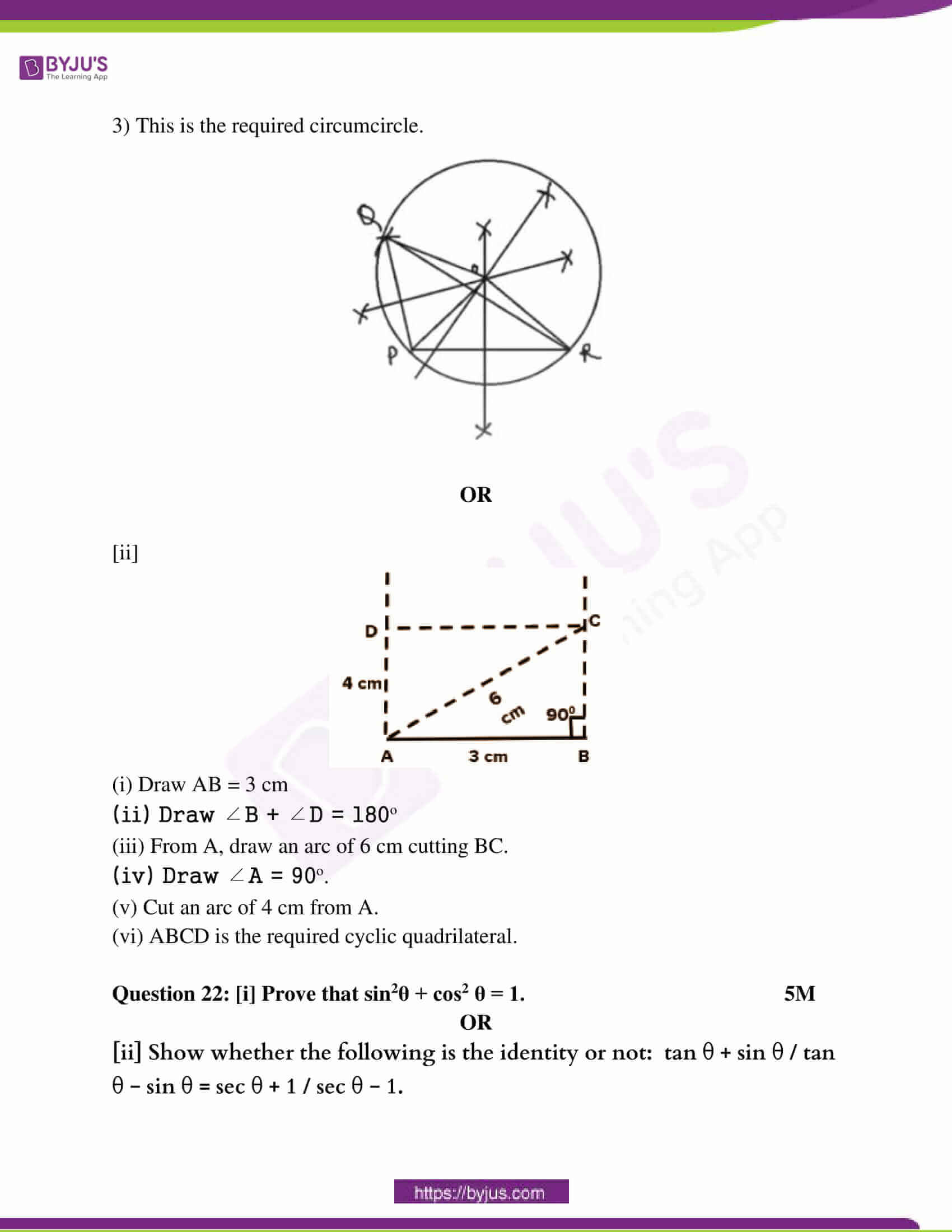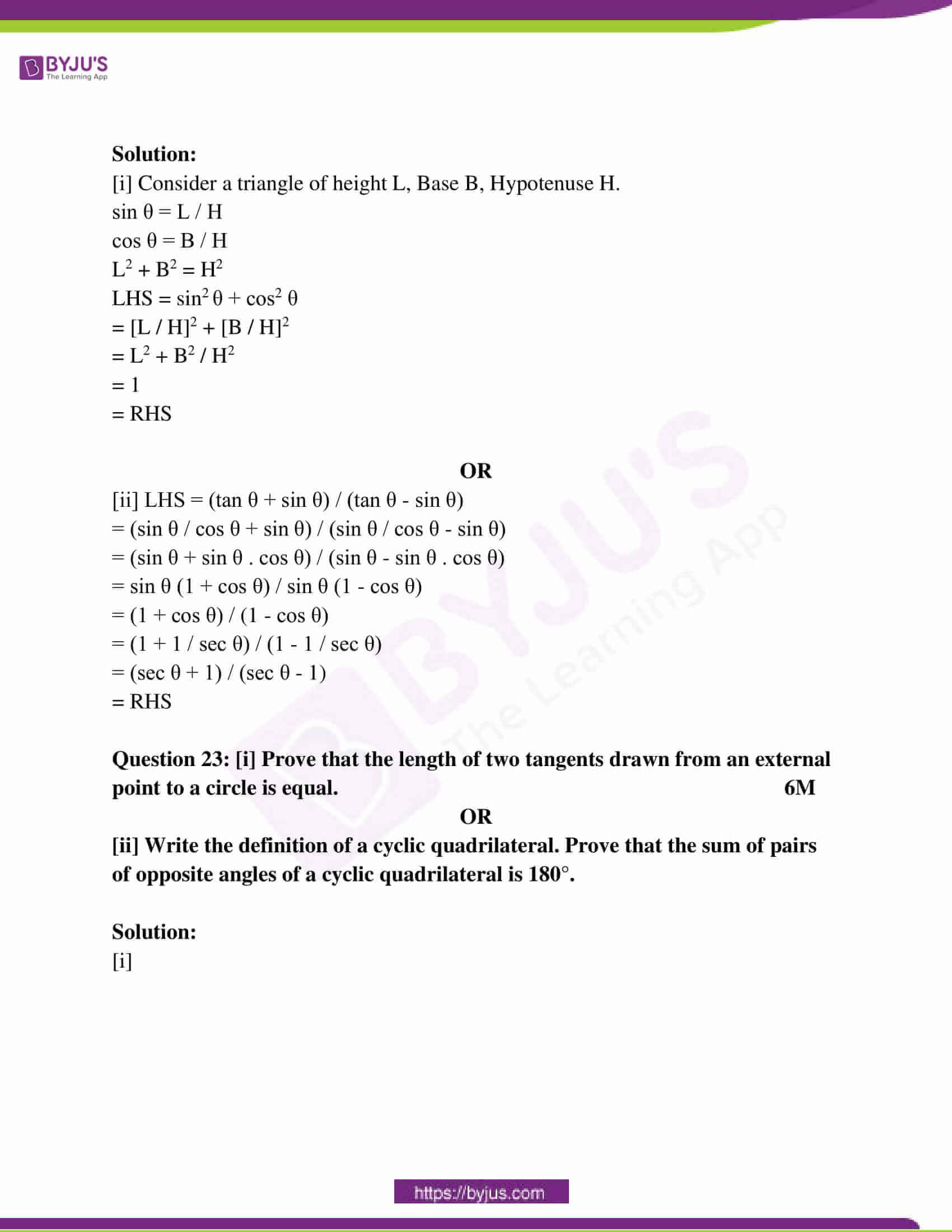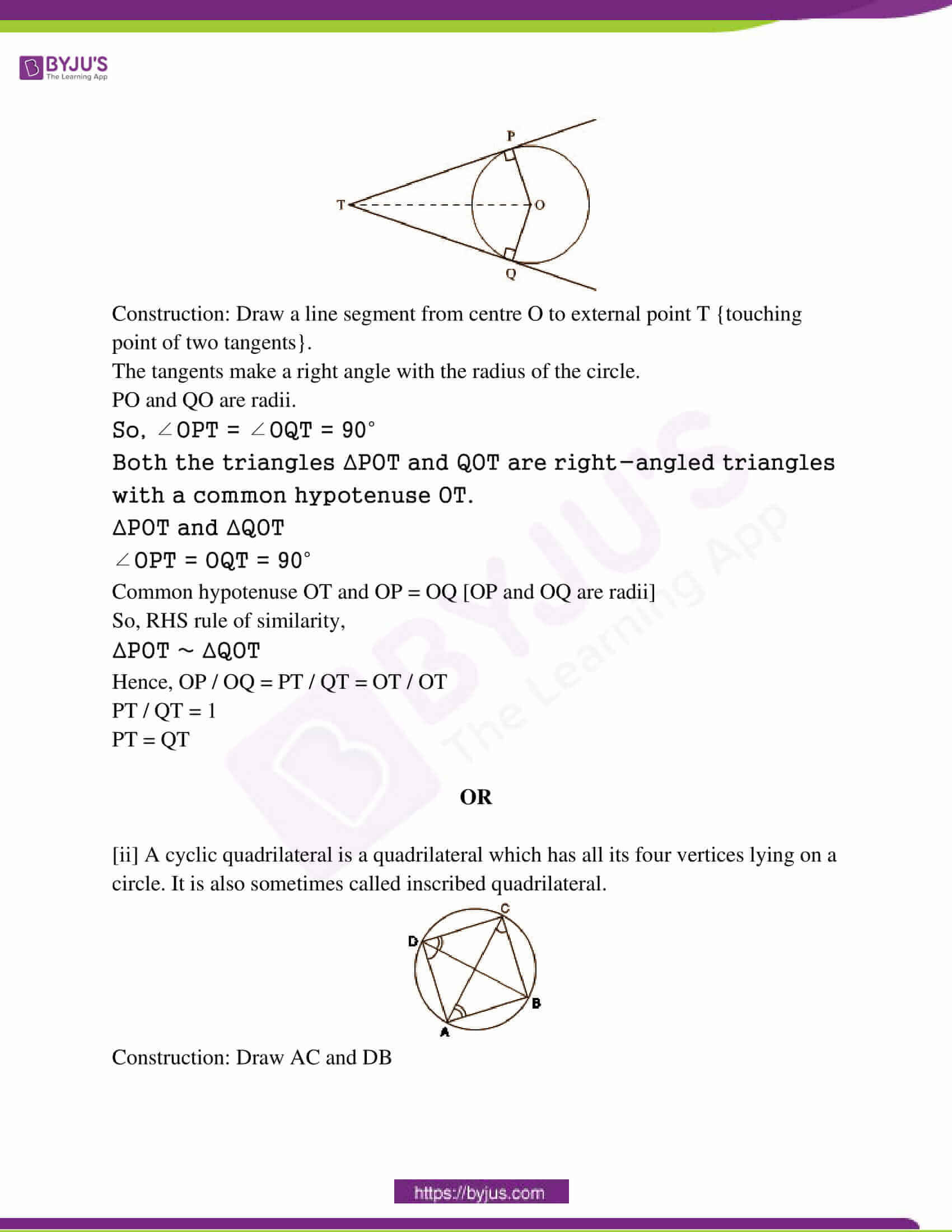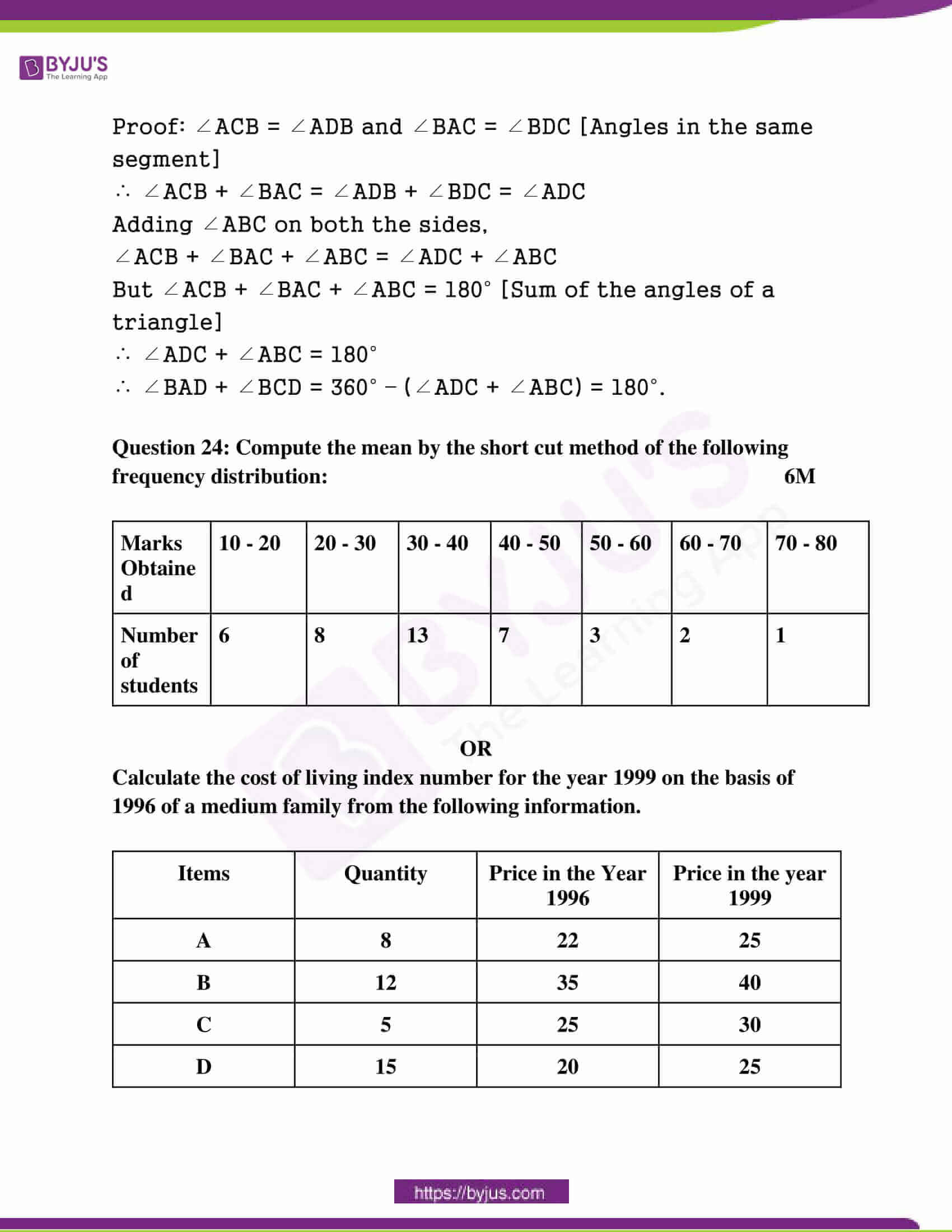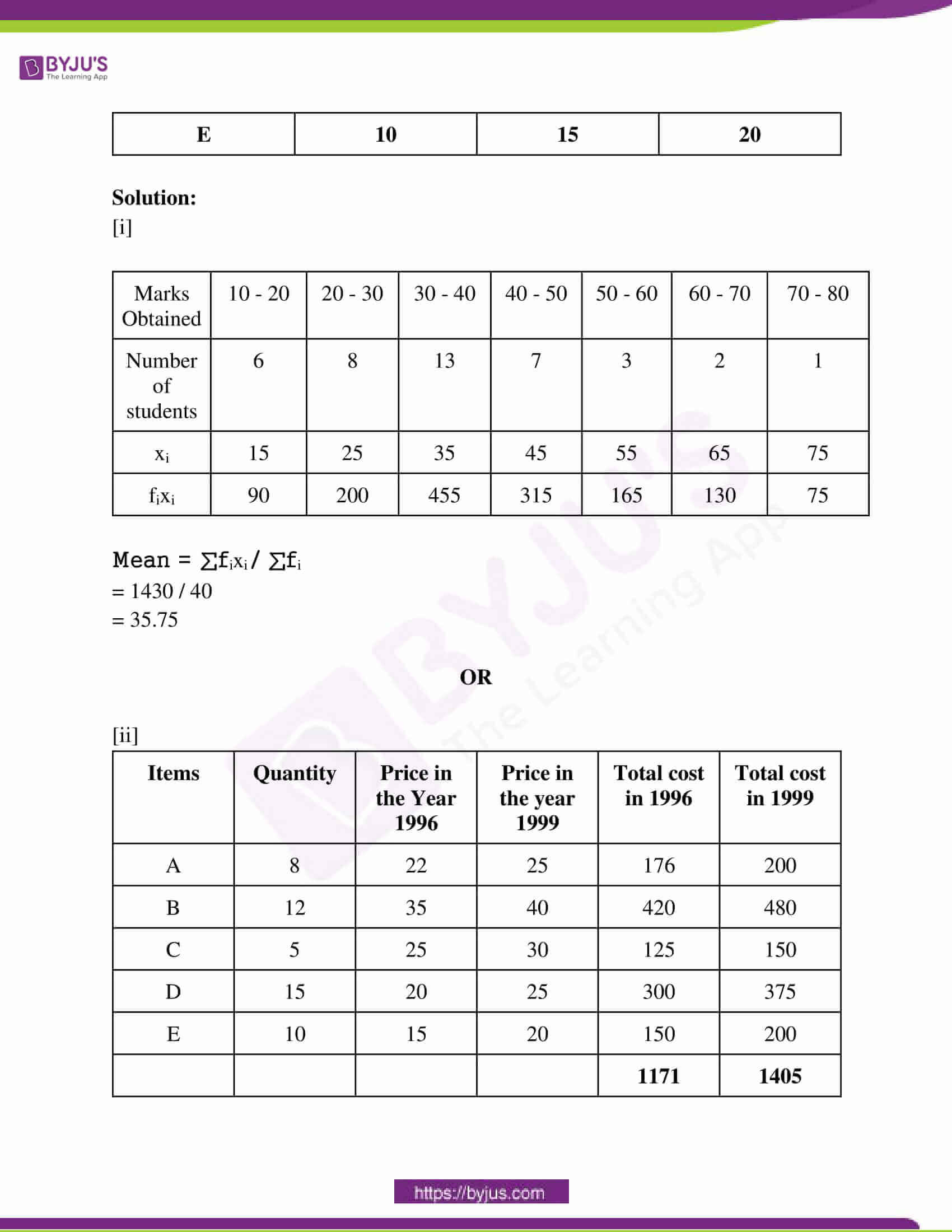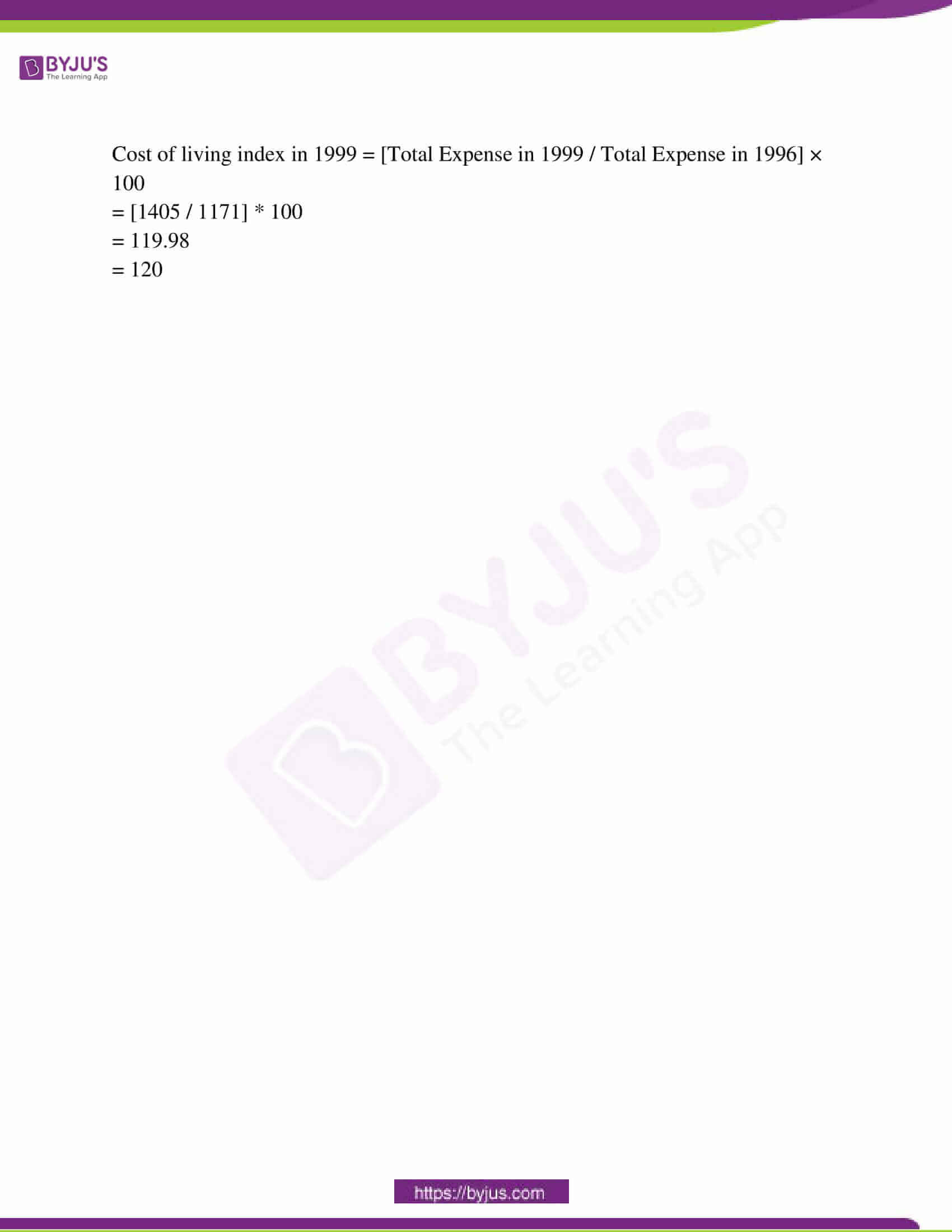Question 1: Choose the correct option and write it in your answer book.

(1 * 5 = 5)

Question i: The system of equations a1x + b1y = c1 and a2x + b2y = c2 represents two parallel lines if,

(a) a1 / a2 = b1 / b2 = c1 / c2 (b) a1 / a2 ≠ b1 / b2

(c) a1 / a2 = b1 / b2 ≠ c1 / c2 (d) a1 / a2 ≠ b1 / b2 = c1 / c2

Question ii: The third proportional to 9, 12 is

(a) 6 √3 (b) 3√6 (c) 27 / 4 (d) 16

Question iii: The maximum degree of the variable used in the quadratic equation is.

(a) 1 (b) 2 (c) 3 (d) 4

Question iv: The measure of ∠PAQ in the figure given below is: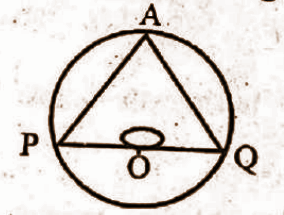(a) 45° (b) 180° (c)90° (d) 60°

Question v: From a point 30 m away from the foot of the building the angle of elevation of the top of the building is 45°. The height of the building will be

(a) 25m (b) 30m (c) 25√2 m (d) 30√2 m

Question 2: Fill in the blanks: 1 * 5 = 5

(i) The zero of the linear polynomial ax + b is ____________ [-b / a]

(ii) The rate of the depreciation is __________ [negative]

(iii) If the sides of two triangles are proportional, then triangles will be ___________ [similar]

(iv) The radius of a circle is 7cm, then the area will be _________________ [154 cm2]

(v) The length of the diagonal of a cube is 12√3 cm. The length of the edge of the cube will be___________. [12 cm]

Question 3: Match the following. 1 * 5 = 5

Column – A Column – B

(i) sin2 25o + cos2 25o (a) cos θ

(ii) 1 + cot2 θ (b) 1

(iii) sin (90° – θ) (c) cosec2θ

(iv) sec 60° (d) tan 41°

(v) tan 49° (e) 2

(f) 1 / 2

(g) cot 41°

(i) – (b)

(ii) – (c)

(iii) – (a)

(iv) – (e)

(v) – (g)

Question 4: Write true/ false in the following: 1 * 5 = 5

(i) X + 2√x is not a polynomial. [True]

(ii) Income tax is a direct tax. [True]

(iii) Angles in the same segment of a circle are equal. [True]

(iv) The volume of a hemisphere is 3𝜋𝑟2. [False]

(v) The probability of a definite event is always one. [True]

Question 5: Write the answer in one word / sentence of each: 1 * 5 = 5

(i) In equation x + 2y = 5, if y = 0 then write the value of x.

x + 2y = 5

x = 5 – 2y

x = 5 – 0

x = 5

(ii) Write the formula of Hero to find the area of a triangle.

√s (s – a) (s – b) (s – c)

(iii) Write the statement of Pythagoras theorem.

Answer: In a right-angle triangle, the square of the hypotenuse is equal to the sum of the square of the other two sides.

(iv) Write the number of circles passing through non-linear points.

(v) Write the value of the mode of the following data: 2, 3, 4, 2, 12, 9, 7, 8, 9, 6, 9, 5, 9.

Question 6: [i] Write the statement of basic proportionality (Thales Theorem).

2M

OR

[ii] Write the property of angle-angle similarity.

Solution:

[i] BPT theorem states that, if a line parallel to a side of a triangle intersects the other sides into two distinct points, then the line divides those sides in equal proportion.

OR

[ii] In two triangles, if two pairs of corresponding angles are equal, then it can be shown that all three pairs of corresponding angles are equal, by angle sum property.

Question 7: [i] The perimeters of two similar triangles are 30cm and 20cm, respectively. If one side of one triangle is 12cm, find the corresponding side of another triangle. 2M

OR

[ii] Triangle ACB is an isosceles triangle such that AC = BC. If AB2 = 2 AC2. Prove that ∆ACB is a right-angled triangle.

Solution:

[i] Let △ABC and △PQR be two similar triangles.

⇒ Perimeter of △ABC = 20 cm.

⇒ Perimeter of △PQR = 30 cm.

⇒ QR = 12 cm, BC = ?

In the similarity case

Perimeter of △ABC / Perimeter of △PQR = AB / PQ = BC / QR = AC / PR

⇒ 20 / 30 = BC / 12

⇒ BC = 8 cm

OR

[ii]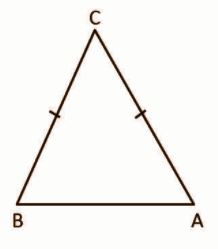AB2 = 2AC2

AB2 = AC2 + AC2

AB2 = AC2 + BC2

AB will be the largest side that is the hypotenuse.

By Pythagoras theorem,

H2 = B2 + P2

AB2 = AC2 + BC2

AB2 = AC2 + AC2

AB2 = AB2

LHS = RHS

Since Pythagoras theorem is satisfied, ∆ACB is a right-angled triangle.

Question 8: [i] Triangle ABC and triangle PQR are two similar triangles. The areas of these are 64 cm2 and 100 cm2, respectively. If QR = 12cm. Then find the value of side BC. 2M

OR

[ii] In the figure given below DE║BC, if AD / DB = 3 / 5 and side AC = 6cm. Then, find the value of AE.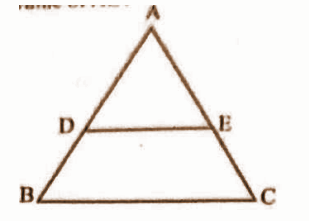Solution:

[i] ΔABC ≈ Δ PQR

Area of Δ ABC / Area of ΔPQR = (AB / PQ)² = (BC / QR)² = (AC / PR)²

Area of Δ ABC = 64 cm²

Area of Δ PQR = 100 cm²

QR = 12 cm

BC = ?

Area of ΔABC / Area of Δ PQR = (BC / QR)²

=> 64 / 100 = (BC / 12)²

=> 16 / 25 = BC² / 122

=> 0.64 = BC2 / 144

=> 92.16 = BC2

=> BC = 9.6 cm

OR

[ii] DE || BC

Using BPT theorem

AD / DB = AE / EC

EC = AC – AE

AD / DB = AE / AC – AE

3 / 5 = AE / 6 – AE

0.6 = AE / 6 – AE

3.6 – 0.6 AE = AE

3.6 = AE + 0.6 AE

3.6 = 1.6AE

AE = 2.25 cm

Question 9: [i] Write any two properties of Arithmetic Mean. 2M

OR

[ii] Find the Median of the following values:

5, 10, 3, 7, 1, 9, 6, 2, 11

Solution:

[i] (a) The sum of deviations of the items from their arithmetic mean is always zero, i.e. ∑(x – X) = 0.

(b) The sum of the squared deviations of the items from Arithmetic Mean (A.M) is minimum, which is less than the sum of the squared deviations of the items from any other values.

OR

[ii] Arrange the data

1, 2, 3, 5, 6, 7, 9, 10, 11

Median = {[n + 1] / 2}th term

= {[9 + 1] / 2}th term

= 5th term

Median = 6

Question 10: [i] Write the probability of getting an odd number in a single throw of a die. 2M

OR

[ii] If two coins are tossed simultaneously, find the probability of getting two heads.

Solution:

[i] Sample space of die = {1, 2, 3, 4, 5, 6}

Odd numbers = {1, 3, 5}

Probability (getting an odd number) = odd numbers / sample space

= 3 / 6

= 1 / 2

OR

[ii] If we toss two coins simultaneously, then possible outcomes (s), are

S = {HT, TH, HH, TT}

⇒ n (S) = 4

Let E be the favourable outcomes of getting two heads, then E = {H, H}

⇒ n (E) = 1

P (getting two heads) = n (E) / n (S)

= 1 / 4

Question 11: [i] Solve the following system of equation 4M

3x – 2y = 4

y + 2x = 5

OR

[ii] Find the value of m, for which the system

2x+ my – 4 = 0

3x – 7y – 10 = 0 has

(i) a unique solution

(ii) no solution

Solution:

[i] 3x – 2y = 4 —- (1)

2x + y = 5 —- (2)

Multiply equation (1) by 2 and equation (2) by 3,

6x – 4y = 8

6x + 3y = 15

___________

-7y = -7

y = 1

Put y = 1 in (2),

2x + y = 5

2x + 1 = 5

2x = 4

x = 4 / 2

x = 2

OR

[ii] 2x + my – 4 = 0

3x – 7y – 10 = 0

Comparing with ax + by + c = 0

a1 = 2, b1 = m, c1 = – 4

a2 = 3, b2 = – 7, c2 = – 10

(1) for unique solution,

a1 / a2 ≠ b1 / b2

2 / 3 = m / -7

-14 = 3m

m = -14 / 3

For all real values of m, [m ≠ -14 / 3] the system has a unique solution.

(2) for no solution, the lines are parallel such that,

a1 / a2 = b1 / b2 ≠ c1 / c2

2 / 3 = m / -7 = -4 / -10

m ≠ [-7 * 4] / -10

m ≠ -14 / 5

So, m takes every value except [-14 / 5].

Question 12: [i] The sum of two numbers is 7. If the sum of these numbers is seven times its difference. Find the number. 4M

OR

[ii] If in ∆ABC ∠C = 2∠B = ∠A + ∠B + 20°, then find all the three angles of a triangle.

Solution:

[i] Let the numbers be x and y.

x + y = 7 ……….. (1)

x + y = 7(x – y)……(2)

x + y = 7x – 7y

0 = 6x – 8y

0 = 3x – 4y………… (3)

Solving (1) and (3)

x + y = 7 × 4

3x – 4y = 0

4x + 4y = 28

__________

7x = 28

x = 4

y = 7 – x (from equation 1)

= 7 – 4

= 3

OR

[ii] Let ∠A be x and ∠B be y.

Given that ∠C = 2∠B

∠C = 2y

Also,

∠C = ∠A + ∠B + 20°

∠C = x + y + 20

Since ABC is a triangle, by angle sum property,

∠A + ∠B + ∠C = 180o

x + y + 2y = 180o

x + 3y = 180o —- (1)

∠A + ∠B + ∠C = 180o

x + y + [x + y + 20] = 180o

x + y + x + y + 20 = 180o

2x + 2y = 160o

x + y = 80o —- (2)

Consider the equations (1) and (2),

x + 3y = 180o

x + y = 80o

_________

2y = 100

y = 100 / 2

y = 50o

∠C = 2y

= 2 * 50o

= 100o

Put y = 50 in (1),

x + 3 * 50 = 180o

x = 180o – 150o

x = 30o

∠A = 30o

∠B = 50o

∠C = 100o

Question 13: [i] If x / [b + c] = y / [c + a] = z / [a + b], then prove that (b – c) x + (c – a) y + (a – b) z = 0. 4M

OR

[ii] What should be subtracted from 11, 20, 26 and 50 so that the remaining numbers are in proportion?

Solution:

[i] Let x / [b + c] = y / [c + a] = z / [a + b] = k

x = (b + c) k …(1)

y = (c + a) k …(2)

z = (a + b) k …(3)

To prove (b – c) x + (c – a) y + (a – b) z = 0

LHS = (b – c) x + (c – a) y + (a – b) z

= (b – c) (b + c) k + (c – a) (c + a) k + (a – b) (a + b) k

= k [(b – c) (b + c) + (c – a) (c + a) + (a – b) (a + b) ]

= k [b2 – c2 + c2 – a2 + a2 – b2]

= k 

= 0

= RHS

OR

[ii] Let the number be subtracted be x so, (11 – x) : (20 – x) : : (26 – x) : (50 – x)

11 – x / 20 – x = 26 – x / 50 – x

(11 – x) (50 – x) = (26 – x) (20 – x)

550 – 11x – 50x + x2 = 520 – 26x – 20x + x2

550 – 61x = 520 – 46 x

550 – 520 = – 46x + 61x

30 = 15x

x = 30 / 15

= 2

Question 14: [i] Solve the equation 3x – 1 / x = 2 using the formula method. 4M

OR

[ii] Find the nature of the roots of the following equation, 6x2 – x – 2 = 0.

Solution:

[i] 3x – [1 / x] = 2

3x2 – 1 = 2x

3x2 – 2x – 1 = 0

a = 3, b = -2, c = -1

x = -b ± √b2 – 4ac / [2a]

= (2) ± √(-2)2 – 4 * 3 * (-1) / [2 * 3]

= 2 ± √16 / 6

= 2 ± 4 / 6

x = 2 + 4 / 6

= 6 / 6

x = 1

x = 2 – 4 / 6

= -2 / 6

= -1 / 3

x = 1, -1 / 3

OR

[ii] The discriminant formula (b2 – 4ac) is used.

• If discriminant > zero, then it has real and unequal roots.
• If discriminant = zero, then it has real and equal roots.
• If discriminant < zero, then it has unreal roots.

6x2 – x – 2 = 0

a = 6 ; b = -1 ; c = -2

Δ = b2 – 4ac

= 1 – 4 * 6 * (-2)

= 1 + 48

= 49

So, Δ > 0

It has real and unequal roots.

Question 15: [i] From the top of the hill, the angle of depression of the top and the bottom of the 16m high building is 30° and 60° respectively. Find the height of the hill. 4M

OR

[ii] An aeroplane is flying at a height of 8,000m. The angle of depression of the control tower of the airport from the aeroplane is 30°. Find the horizontal distance between the control tower and the aeroplane.

Solution:

[i] Let the height of building ‘h’ m = AD

Height of the tall building = 16 m = BE

The angle of depression of top of the tall building from the multistoried building = 30°

The angle of depression of bottom of the tall building from the multistoried building = 60°

So, BC = x and CD = 16 m [As BCDE forms a rectangle]

So, AC = (h – 16) m

In ΔBCA

tan 30o = AC/ BC

1 / √3 = (h – 16)/x

x = √3(h – 16)…… (i)

tan 60o = AD / ED

1.732 = h / x

h = x

h = √3(h – 16) [using (i)]

h = √3h – 16√3

(√3 – 1)h = 16√3

h = 16√3/ (√3 -1)

Rationalising the denominator by (√3 + 1), we have

h = 16√3(√3 + 1) / (3 – 1)

h = 16√3(√3 + 1) / 2

h = 48 + 16 / 2

= 64 / 2

= 32m

The height of the building is 32m.

OR

[ii] Let the horizontal distance between the control tower and the aeroplane be x.

tan 30o = 8000 / x

x = 8000 / tan 30o

x = 13856.4m

Question 16: [i] A quadrants shaped flower bed is made of radius 14m in a square garden of side 100m, in all the four corners. Find the area of the remaining part of the square garden. 4M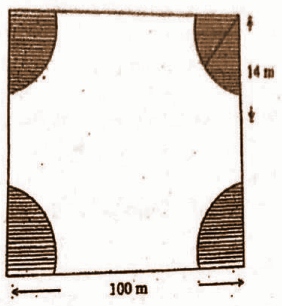OR

[ii] A rocket is in the form of a closed cylinder from below, and the upper part surmounted by a cone of equal radius. The radius of the cylinder is 2 m and the height is 21 m. The height of the cone is 8.4m. Find the volume of the rocket.

Solution:

[i] Side of square = 100cm

Angle measure, θ = 90° (angle of each corner of the square is 90°)

Area of square = (side)²

= (100)²

= 10000 cm²

Area of each quadrant = [1 / 4] * 𝛑 * r²

= 1 / 4 * 22 / 7 * 14 *14

= 154cm²

Area of remaining part of square = Area of the square – 4 * Area of each quadrant

= 10000 – 4 * 154

= 10000 – 616

= 9384 cm²

OR

[ii] Volume of Rocket = Volume of cone + Volume of cylinder

= [1 / 3]𝛑r2h + 𝛑R2h

= [1 / 3] * [22 / 7] * 22 * (8.4) + [22 / 7] * 22 * 21

= 35.2 + 264

= 299.2m3

Question 17: [i] The area of three adjacent faces of a cuboid are x, y and z. If the volume of the cuboid is V, then prove that V2 = xyz. 4M

OR

[ii] An iron sphere of radius 8 cm is melted then recast into small spheres each of radius 1 cm. Find the number of small spheres.

Solution:

[i] Let the dimensions of the cuboid are

Length = l

Height = h

Given the area of three faces x, y and z are

lb = x –(1)

bh = y —(2)

lh = z —(3)

Multiply (1), (2) and (3)

lb × bh × lh = xyz

l2 × b2 × h2 = xyz

(lbh)2 = xyz

V2 = xyz [Since the volume of the cuboid = V = lbh]

OR

[ii] Radius of bigger sphere (R) = 8 cm

Radius of small spheres (r) = 1 cm

Let the number of small spheres = n

n × volume of a small spheres = volume of bigger sphere

n * [4 / 3]𝛑r3 = [4 / 3] 𝛑R3

n = R3 / r3

= 83 / 13

= 512

Question 18: [i] Find cyclic factors ab (a – b) + bc (b – c) + ca (c – a). 5M

OR

[ii] Multiply the rational expressions x2 − 7x + 10 / (x − 4)2 and x2 − 7x + 12 / x − 5 and express the product in its lowest terms.

Solution:

[i] ab (a – b) + bc (b – c) + ca (c – a)

a2 b − ab2 + b2c − bc2 + ac2 − a2c

= a2(b − c) − a (b − c) (b + c) + bc (b − c)

= (b − c) [a2 − a (b + c) + bc]

= (b − c) [a2 − ab − ac + bc]

= (b − c) [a (a − b) − c (a − b)]

= (b − c) (a − b) (a − c)

OR

[ii]{x2 – 7x + 10}/ {(x – 4)2}* {x2 – 7x + 12} / {x – 5}

= [x2 – 5x – 2x – 10] / (x – 4)2 * (x2 – 4x – 3x – 12) / (x – 5)

= (x – 5) (x – 2) / (x – 4)2 * (x – 4) (x – 3) / (x – 5)

= (x – 2) (x – 3) / (x – 4)

Question 19: [i] If α and β are the roots of quadratic equations 3x2 – 5x – 7 = 0, then find the value of α / β + β / α. 5M

OR

[ii] The length of the side forming the right angle of a right angled triangle are x cm and (x + 1) cm. If the area of the triangle is 10 cm2, then find the sides of the triangle.

Solution:

[i] 3x2 – 5x – 7 = 0

a = 3 ; b = -5 ; c = -7

α + β = -b / a = 5 / 3

αβ = c / a = -7 / 3

α / β + β / α = α2 + β2 / αβ

= (α + β)2 – 2αβ / αβ

= [(5 / 3)2 – 2 * (-7 / 3)] / [-7 / 3]

= [(25 / 9) + (14 / 3) / [-7 / 3]

= [67 / 9] / [-7 / 3]

= -67 / 21

OR

[ii] Area of a triangle = (1 / 2) * bh

[1 / 2] (x (x + 1)) = 10

[1 / 2] [x2 + x] = 10

x2 + x – 20 = 0

x² + x = 20

x² + 2 × x × 1 / 2 = 20

x² + 2 × x × 1 / 2 + (1 / 2)² = 20 + (1 / 2)²

( x + 1 / 2 )² = 20 + 1 / 4

( x + 1 / 2 )² = ( 80 + 1 ) / 4

( x + 1 / 2 )² = 81 / 4

x + 1 / 2 = ± √( 81 / 4 )

x + 1 / 2 = ± 9 / 2

x = -1 / 2 ± 9 / 2

x = ( -1 / 2 + 9 / 2 ) or x = ( -1 / 2 – 9 / 2 )

x = 8 / 2 or x = -10 / 2

x = 4 or x = -5

Hence, sides are 4 cm and 5 cm.

Question 20: [i] Find the compound interest on Rs, 2000 at the rate of interest 4% per annum for 2 years. 5M

OR

[ii] A sewing machine is available for Rs. 1600 Cash or for Rs. 1200 cash down payment and Rs. 460 to be paid after 6 months. Find the rate of interest charged under the instalment plan.

Solution:

[i] Principle = Rs 2000 = P

Interest rate = 4% per annum = R

Time = 2 Years = n

Amount = P (1 + R / 100)ⁿ

=> Amount = 2000 ( 1 + 4 / 100)²

=> Amount = 2000 * 1.04²

=> Amount = 2,163.2

Compound Interest = Amount – Principal

= 2163.2 – 2000

= 163.2 Rs

163.2 Rs is the compound interest on rupees 2000 for 2 years at 4% per annum compounded annually.

OR

[ii] Cash price of sewing machine = 1600 Rs

Down payment = 1200 Rs

Balance due = 1600 – 1200 = 400 Rs

No. of instalment = 1

Amount of instalment = 460 Rs

Interest paid = 460 – 400 = 60 Rs

SI = PRT / 100

60 = [1600 * R * 6] / [100 * 12]

R = 7.5 %

Question 21: [i] The side of the triangle is 4cm, 6cm and 8cm. Draw the circumcircle of the triangle and write the steps of construction. 5M

OR

[ii] Construct a cyclic quadrilateral in which AC = 6cm, ∠B = 90°, AB = 3cm and AD = 4cm. Write the steps for construction also.

Solution:

[i] Steps for construction:

I) Construction of triangle

1) Let the base of the triangle be QR=8cm.

2) Taking Q as the centre and radius 4cm on the compass, draw an arc on the upper side of QR.

3) Taking R as the centre and radius 6cm on the compass, draw an arc on the upper side of QR, intersecting the previous arc. Mark this point of intersection as point P.

4) Join points P to Q and P to Q to complete the △PQR.

II) Construction of perpendicular bisectors

1) Taking Q as the centre and radius more than half of QR, mark arcs below and above the line.

2) Now, with R as the centre and same radius, draw arcs above and below the line to intersect the already drawn arcs. Name the new points as X and Y.

3) Join points X and Y. This line XY is the required perpendicular bisector of side QR.

4) Similarly, draw perpendicular bisectors of sides PQ and PR.

III) Construction of circumcircle

1) All the perpendicular bisectors of △PQR will intersect at one point in the interior of the triangle.

Name that point as O.

2) Taking point O as the centre and OP as the radius draw a circle. Points Q and R should also lie on this circle.

3) This is the required circumcircle.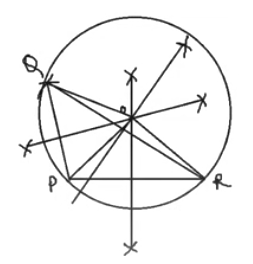OR

[ii]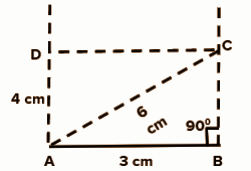(i) Draw AB = 3 cm

(ii) Draw ∠B + ∠D = 180o

(iii) From A, draw an arc of 6 cm cutting BC.

(iv) Draw ∠A = 90o.

(v) Cut an arc of 4 cm from A.

(vi) ABCD is the required cyclic quadrilateral.

Question 22: [i] Prove that sin2θ + cos2 θ = 1. 5M

OR

[ii] Show whether the following is the identity or not: tan θ + sin θ / tan θ − sin θ = sec θ + 1 / sec θ − 1.

Solution:

[i] Consider a triangle of height L, Base B, Hypotenuse H.

sin θ = L / H

cos θ = B / H

L2 + B2 = H2

LHS = sin2 θ + cos2 θ

= [L / H]2 + [B / H]2

= L2 + B2 / H2

= 1

= RHS

OR

[ii] LHS = (tan θ + sin θ) / (tan θ – sin θ)

= (sin θ / cos θ + sin θ) / (sin θ / cos θ – sin θ)

= (sin θ + sin θ . cos θ) / (sin θ – sin θ . cos θ)

= sin θ (1 + cos θ) / sin θ (1 – cos θ)

= (1 + cos θ) / (1 – cos θ)

= (1 + 1 / sec θ) / (1 – 1 / sec θ)

= (sec θ + 1) / (sec θ – 1)

= RHS

Question 23: [i] Prove that the length of two tangents drawn from an external point to a circle is equal. 6M

OR

[ii] Write the definition of a cyclic quadrilateral. Prove that the sum of pairs of opposite angles of a cyclic quadrilateral is 180°.

Solution:

[i]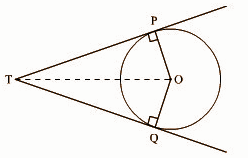Construction: Draw a line segment from centre O to external point T {touching point of two tangents}.

The tangents make a right angle with the radius of the circle.

So, ∠OPT = ∠OQT = 90°

Both the triangles ∆POT and QOT are right-angled triangles with a common hypotenuse OT.

∆POT and ∆QOT

∠OPT = OQT = 90°

Common hypotenuse OT and OP = OQ [OP and OQ are radii]

So, RHS rule of similarity,

∆POT ~ ∆QOT

Hence, OP / OQ = PT / QT = OT / OT

PT / QT = 1

PT = QT

OR

[ii] A cyclic quadrilateral is a quadrilateral which has all its four vertices lying on a circle. It is also sometimes called inscribed quadrilateral.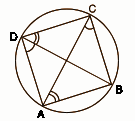Construction: Draw AC and DB

Proof: ∠ACB = ∠ADB and ∠BAC = ∠BDC [Angles in the same segment]

Adding ∠ABC on both the sides,

∠ACB + ∠BAC + ∠ABC = ∠ADC + ∠ABC

But ∠ACB + ∠BAC + ∠ABC = 180° [Sum of the angles of a triangle]

∴ ∠ADC + ∠ABC = 180°

∴ ∠BAD + ∠BCD = 360° – (∠ADC + ∠ABC) = 180°.

Question 24: Compute the mean by the short cut method of the following frequency distribution: 6M

 Marks Obtained 10 – 20 20 – 30 30 – 40 40 – 50 50 – 60 60 – 70 70 – 80 Number of students 6 8 13 7 3 2 1

OR

Calculate the cost of living index number for the year 1999 on the basis of 1996 of a medium family from the following information.

 Items Quantity Price in the Year 1996 Price in the year 1999 A 8 22 25 B 12 35 40 C 5 25 30 D 15 20 25 E 10 15 20

Solution:

[i]
 Marks Obtained 10 – 20 20 – 30 30 – 40 40 – 50 50 – 60 60 – 70 70 – 80 Number of students 6 8 13 7 3 2 1 xi 15 25 35 45 55 65 75 fixi 90 200 455 315 165 130 75

Mean = ∑fixi / ∑fi

= 1430 / 40

= 35.75

OR

[ii]
 Items Quantity Price in the Year 1996 Price in the year 1999 Total cost in 1996 Total cost in 1999 A 8 22 25 176 200 B 12 35 40 420 480 C 5 25 30 125 150 D 15 20 25 300 375 E 10 15 20 150 200 1171 1405

Cost of living index in 1999 = [Total Expense in 1999 / Total Expense in 1996] × 100

= [1405 / 1171] * 100

= 119.98

= 120